# Inter maths solutions for Permutations and Combinations

Text book exercises solutions for Permutations and Combinations second inter maths IIA.

These solutions are very easy to understand.

Study the text book lesson Permutations and Conbinations. Observe the example problems and solutions in the text book.

Try them well and observe the given beliw solutions and try them in your own method.

Exercises 5(a), 5(b), 5(c), 5(d) and 5(e) solutions are given.

You can also see the solutions for

Complex numbers solutions for textbook

De Moivre’s Theorem textbook solutions

Exercise 3(b)

Exercise 3(c)

You can see solutions for Inter Maths IIB

1. Circle

3. Parabola

4. Ellipse

You can see the solutions for first year maths 1A and 1B for examination purpose

Inter maths 1A solutions

3. Matrices

You can also see the solutions for 1b

1. Locus

The straight line sa

Straight lines la

# Permutations and Combinations solutions

Exercise  (5a)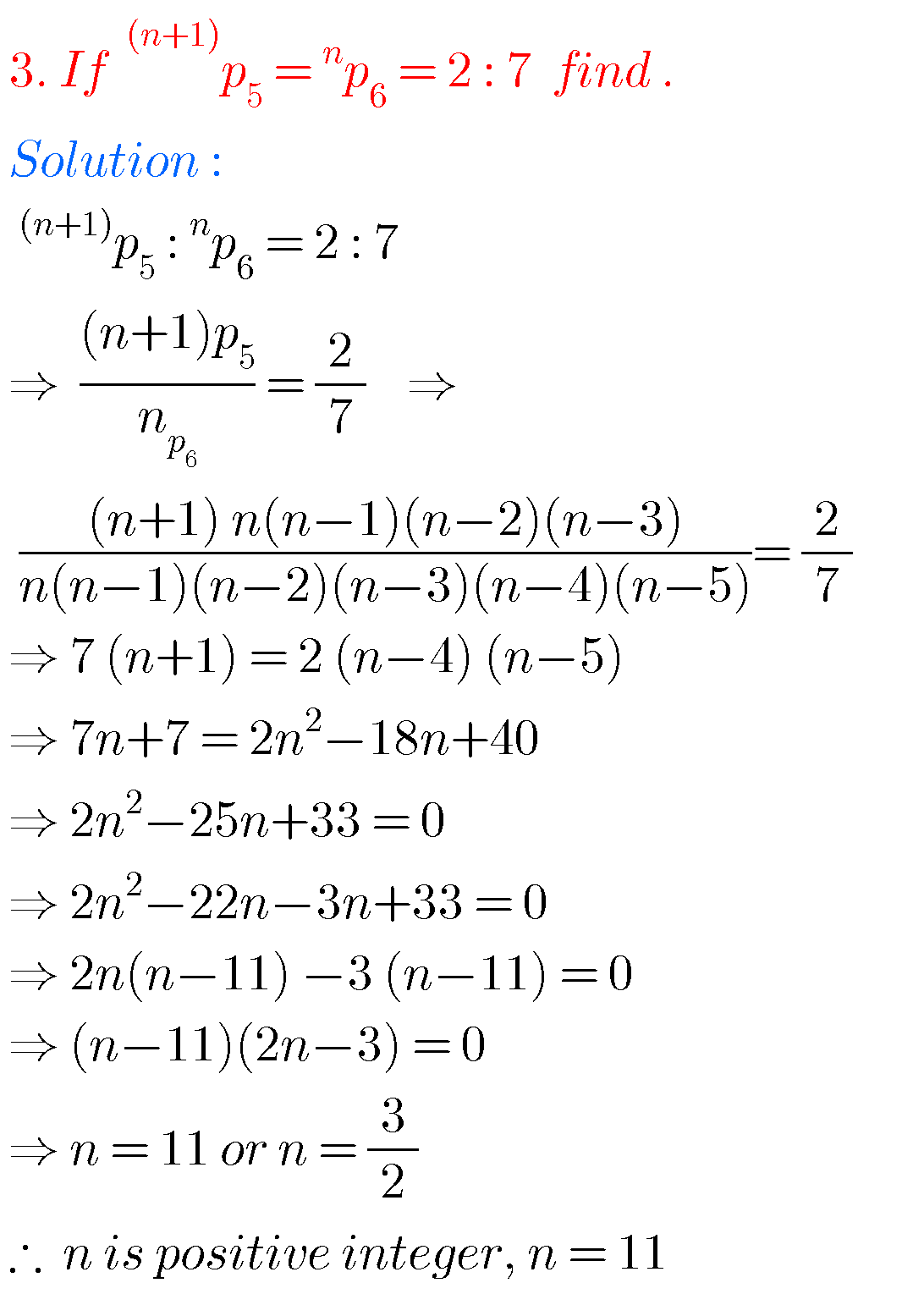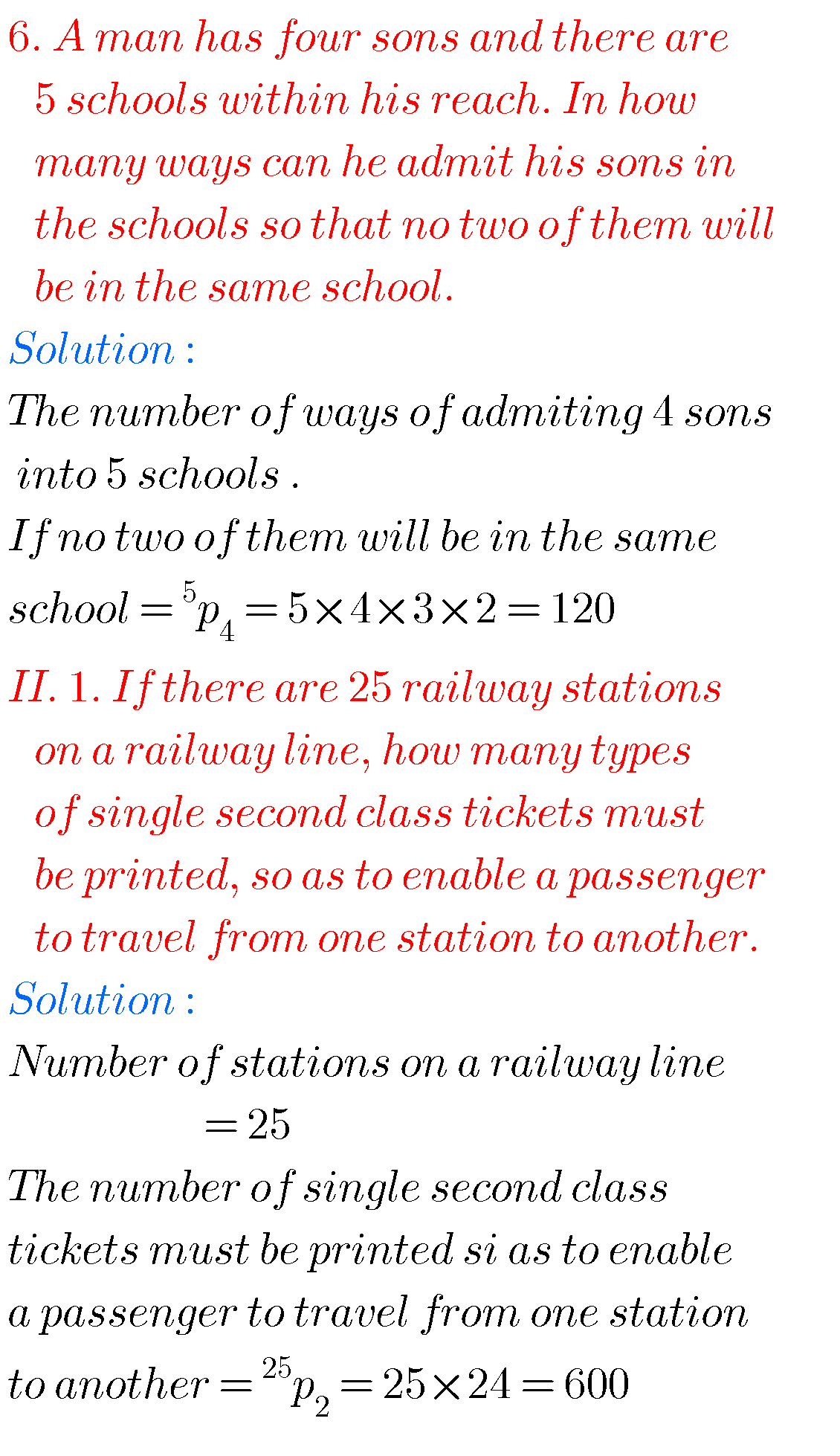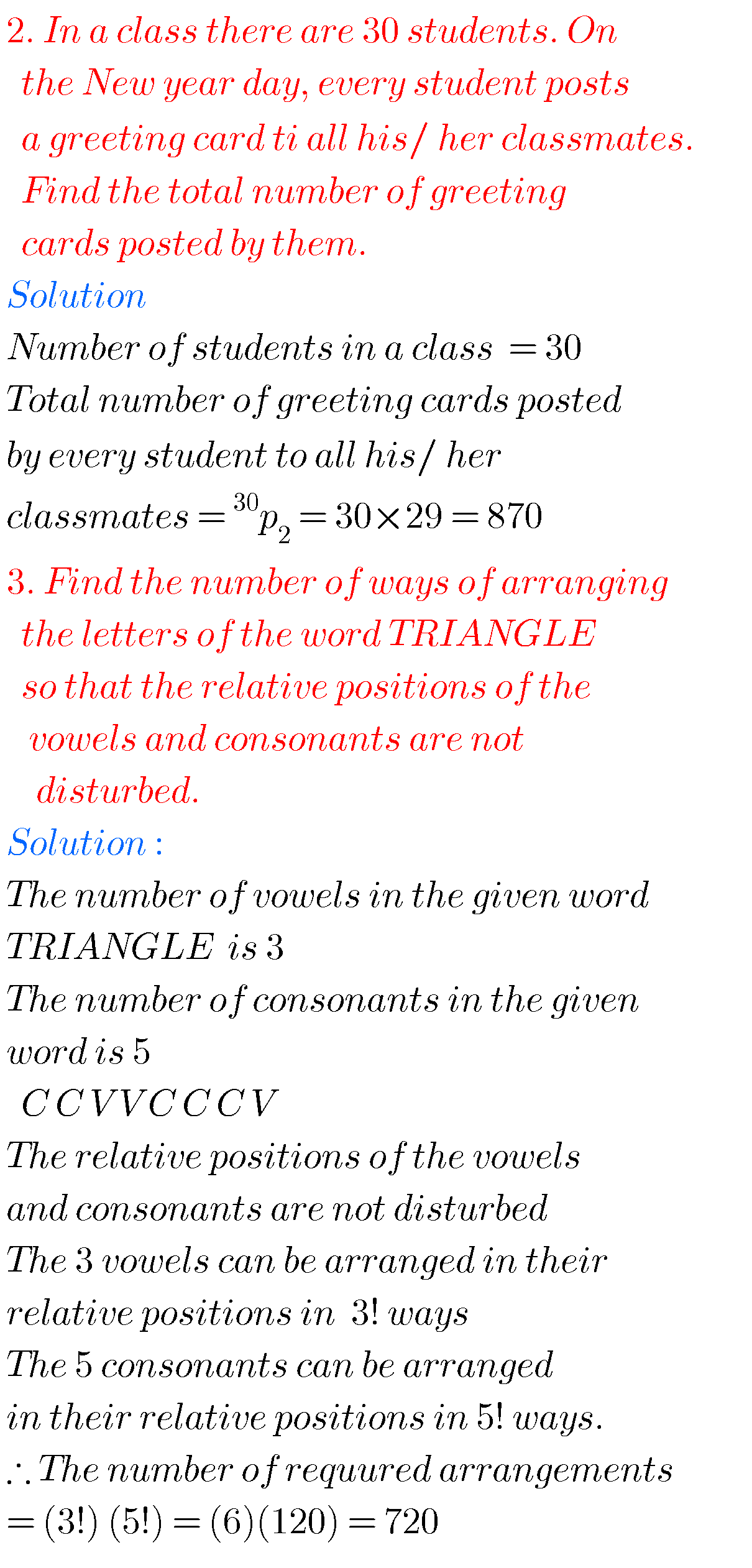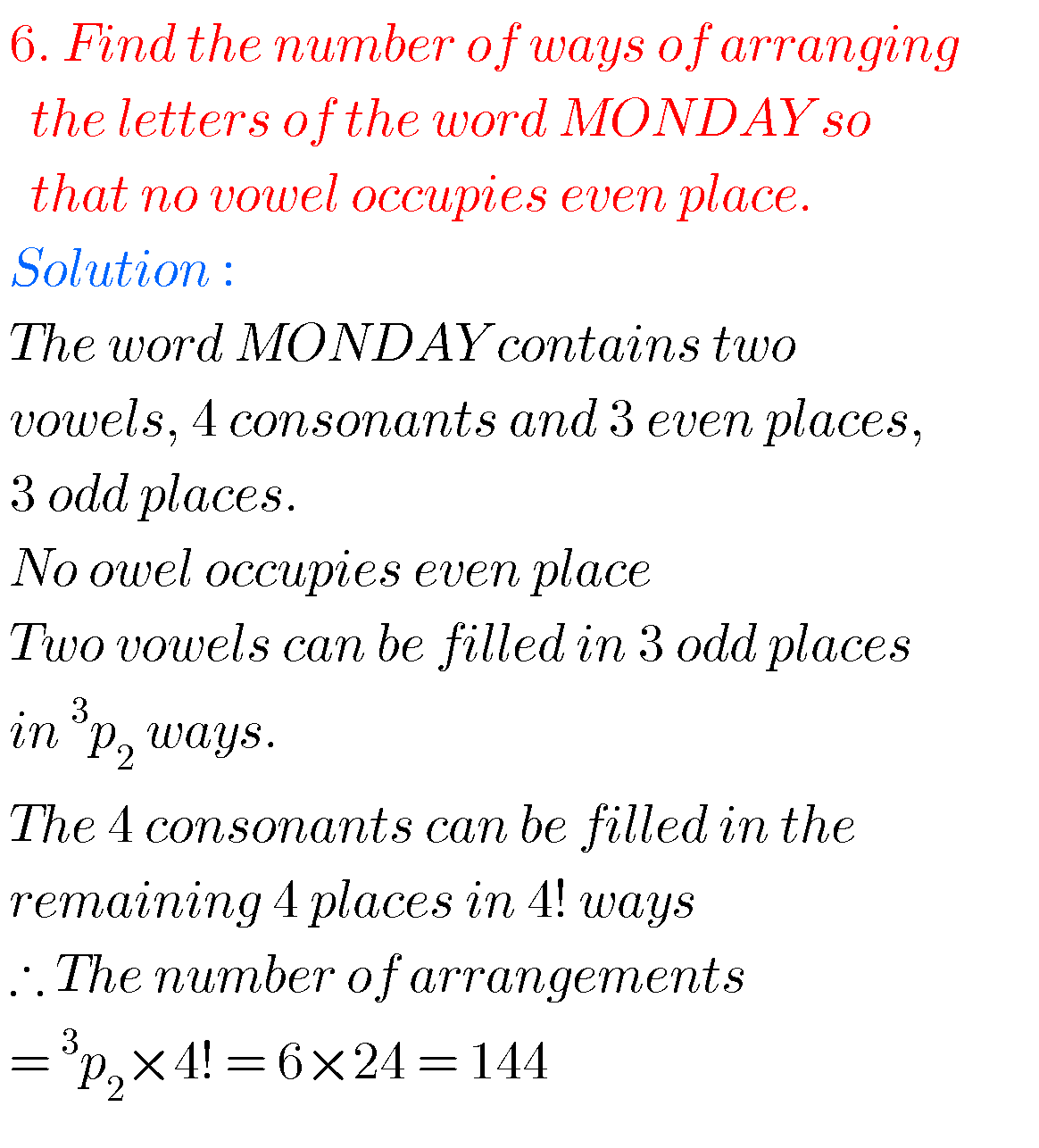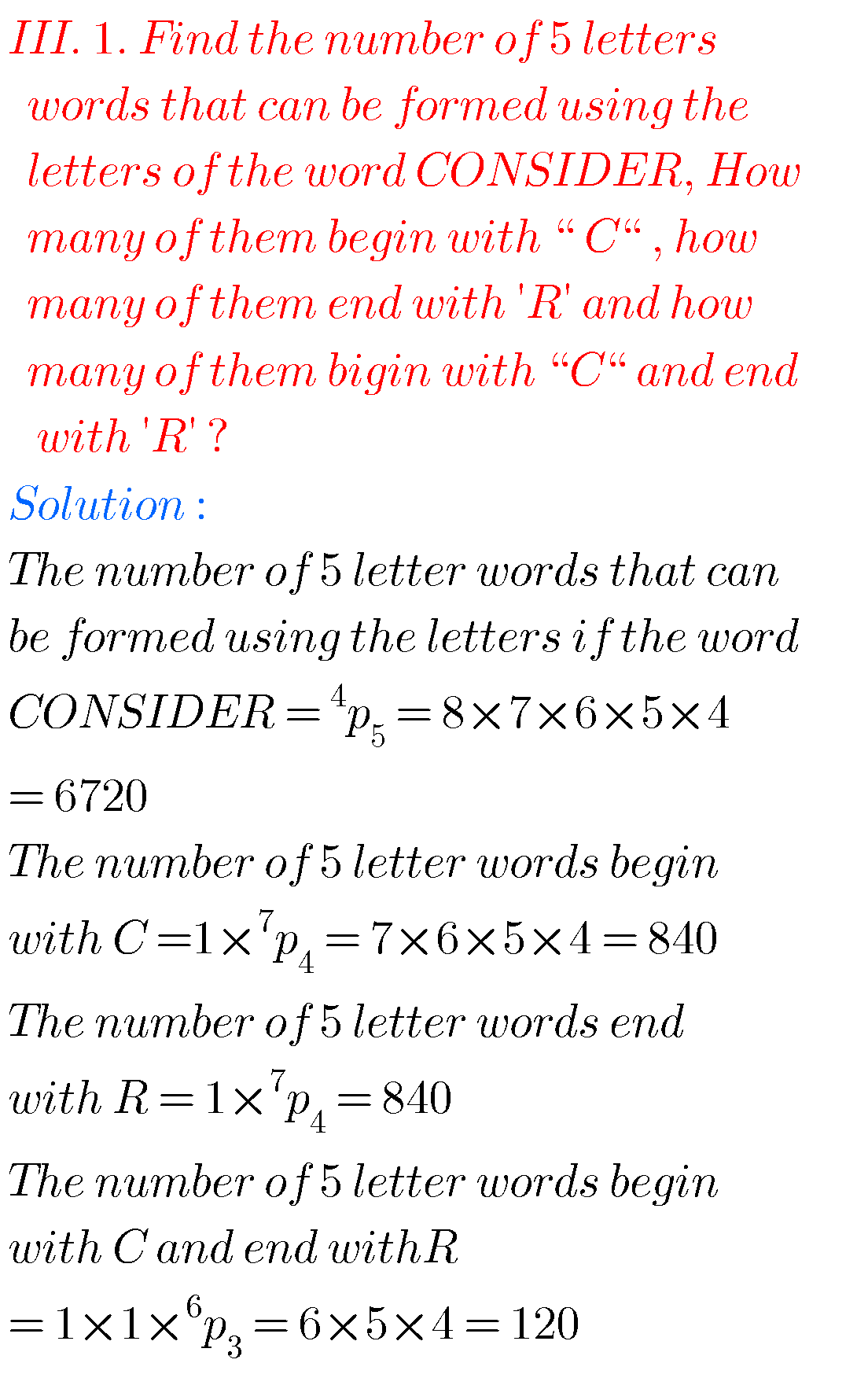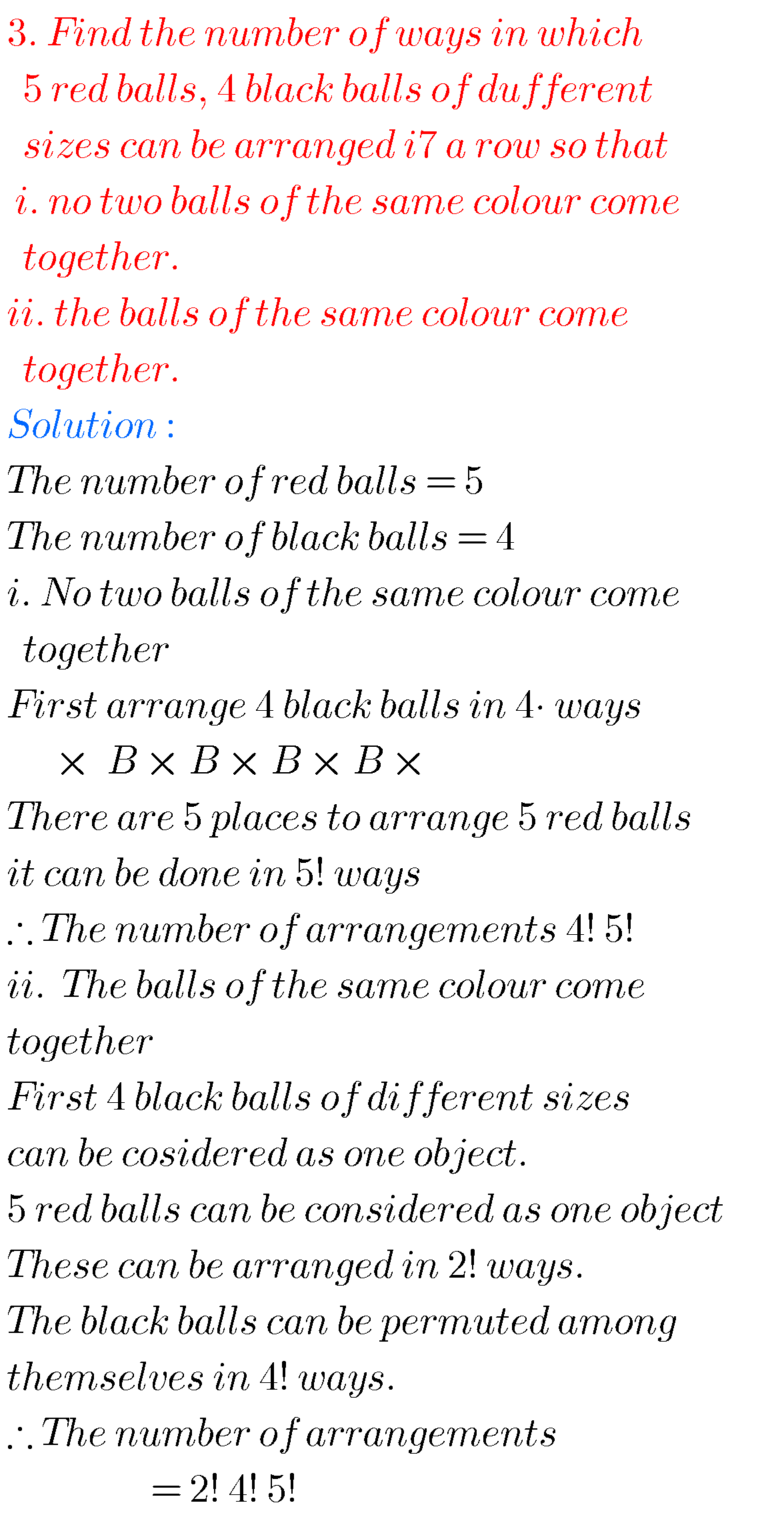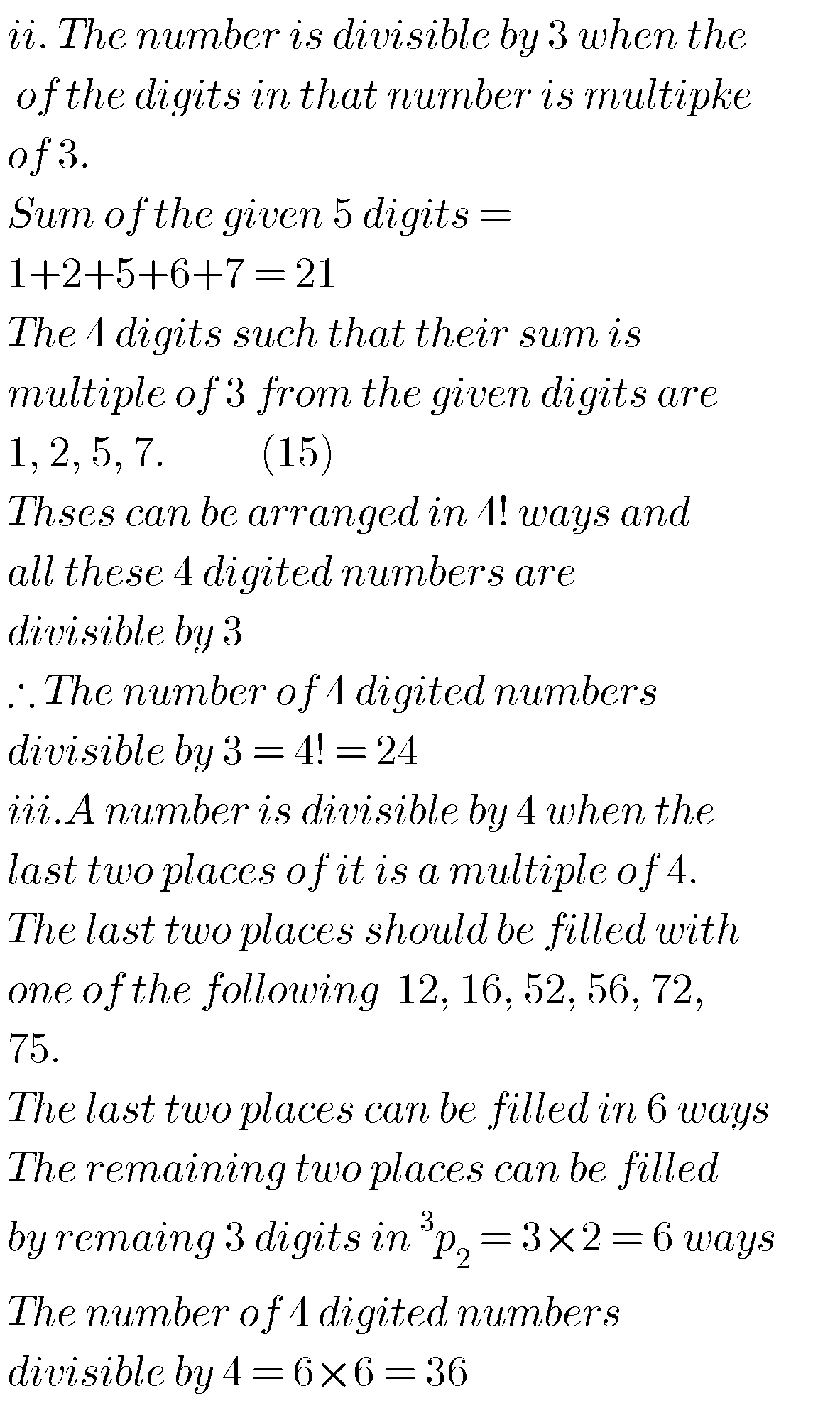## Solutions for Permutations and Combinations

Exercise 5(b)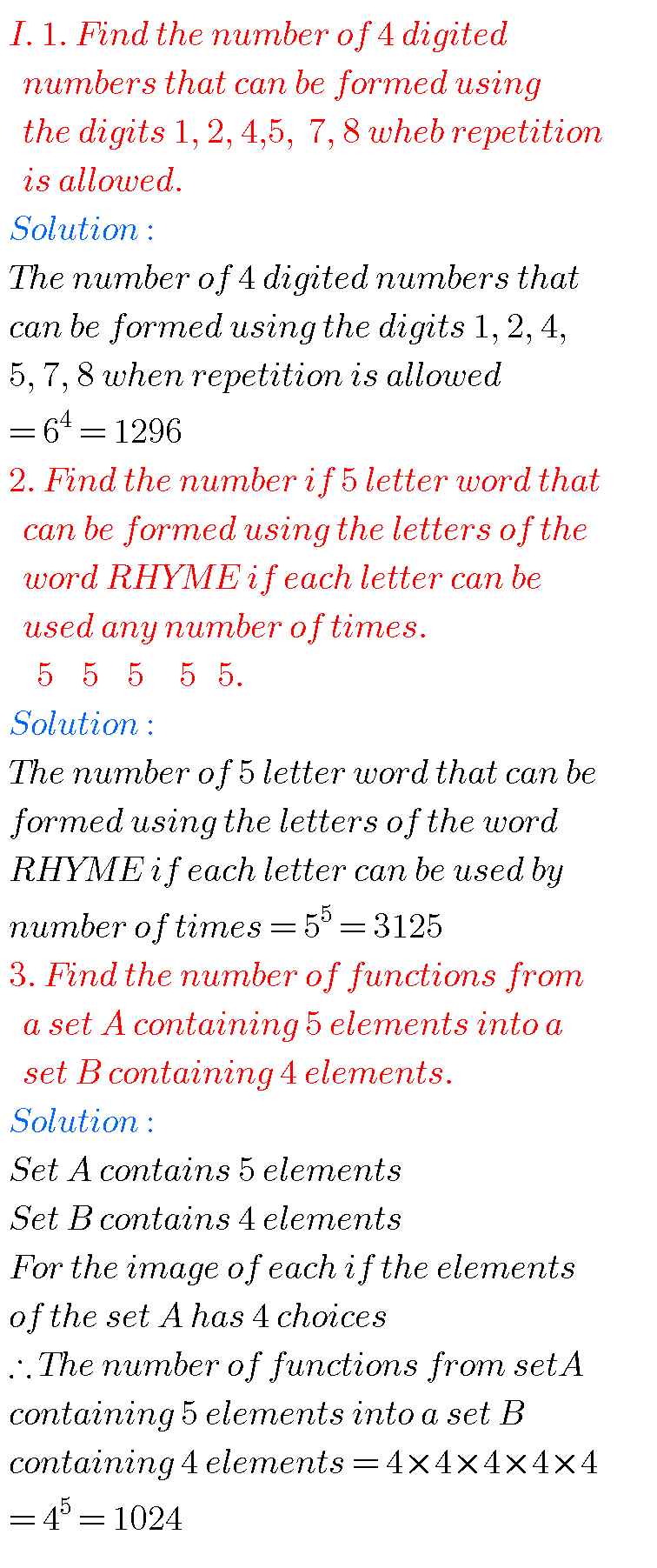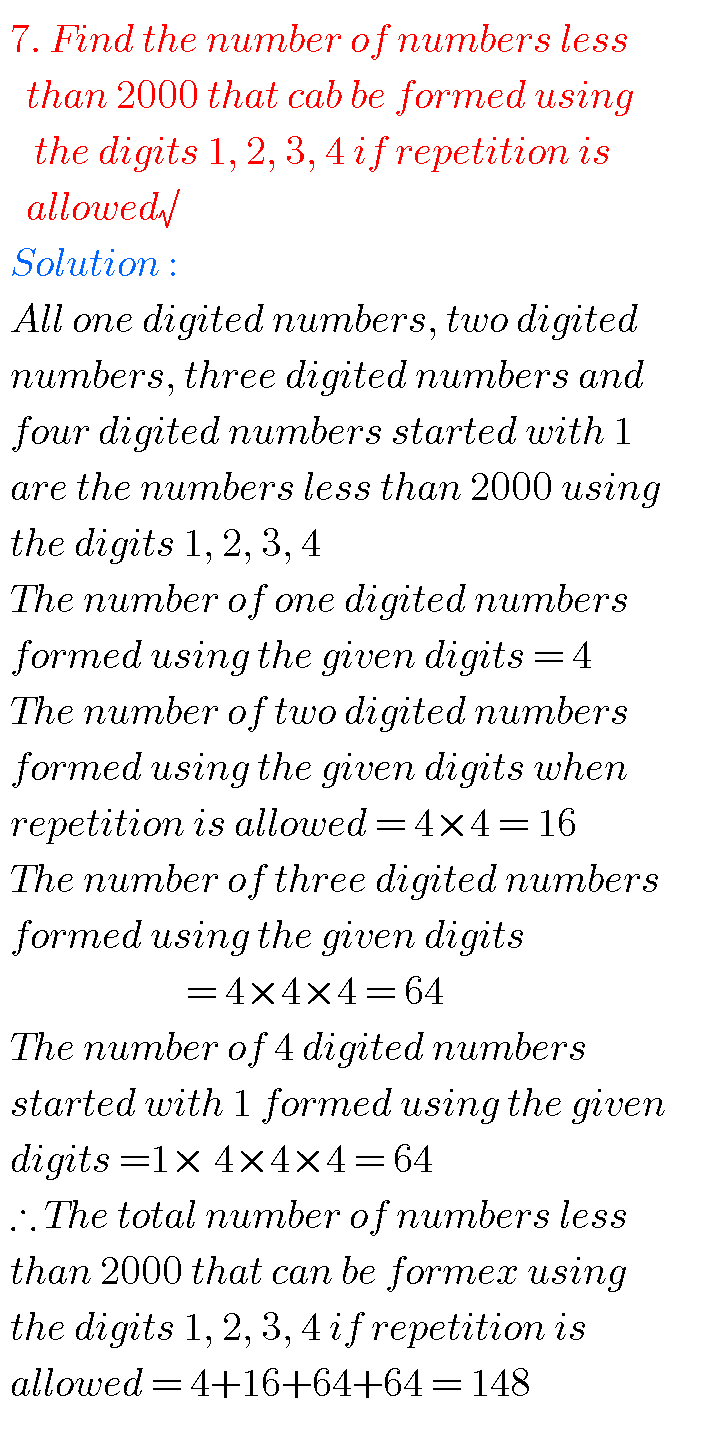## Maths solutions for Permutations and Combinations inter

Exercise 5(c)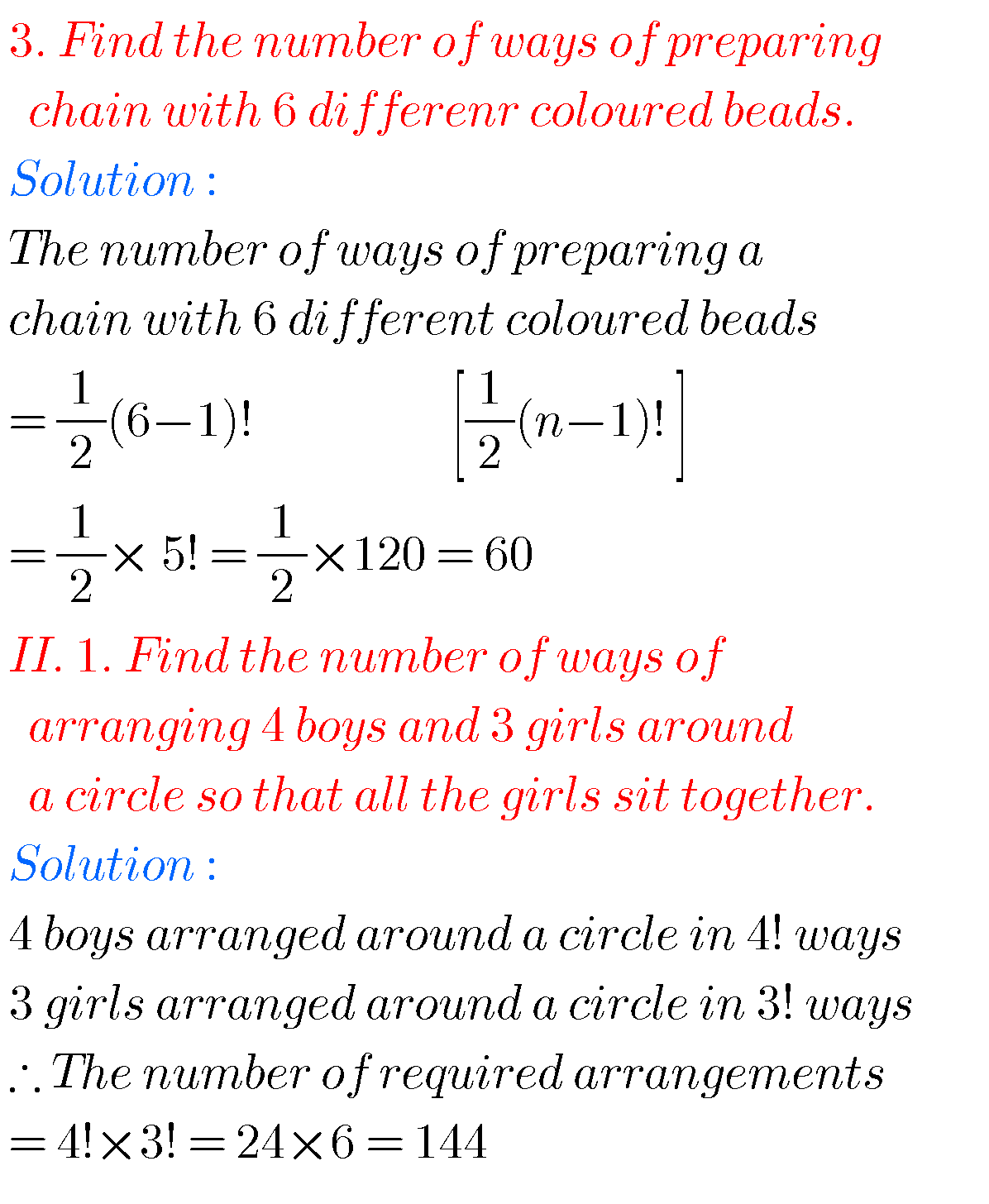Figure for 2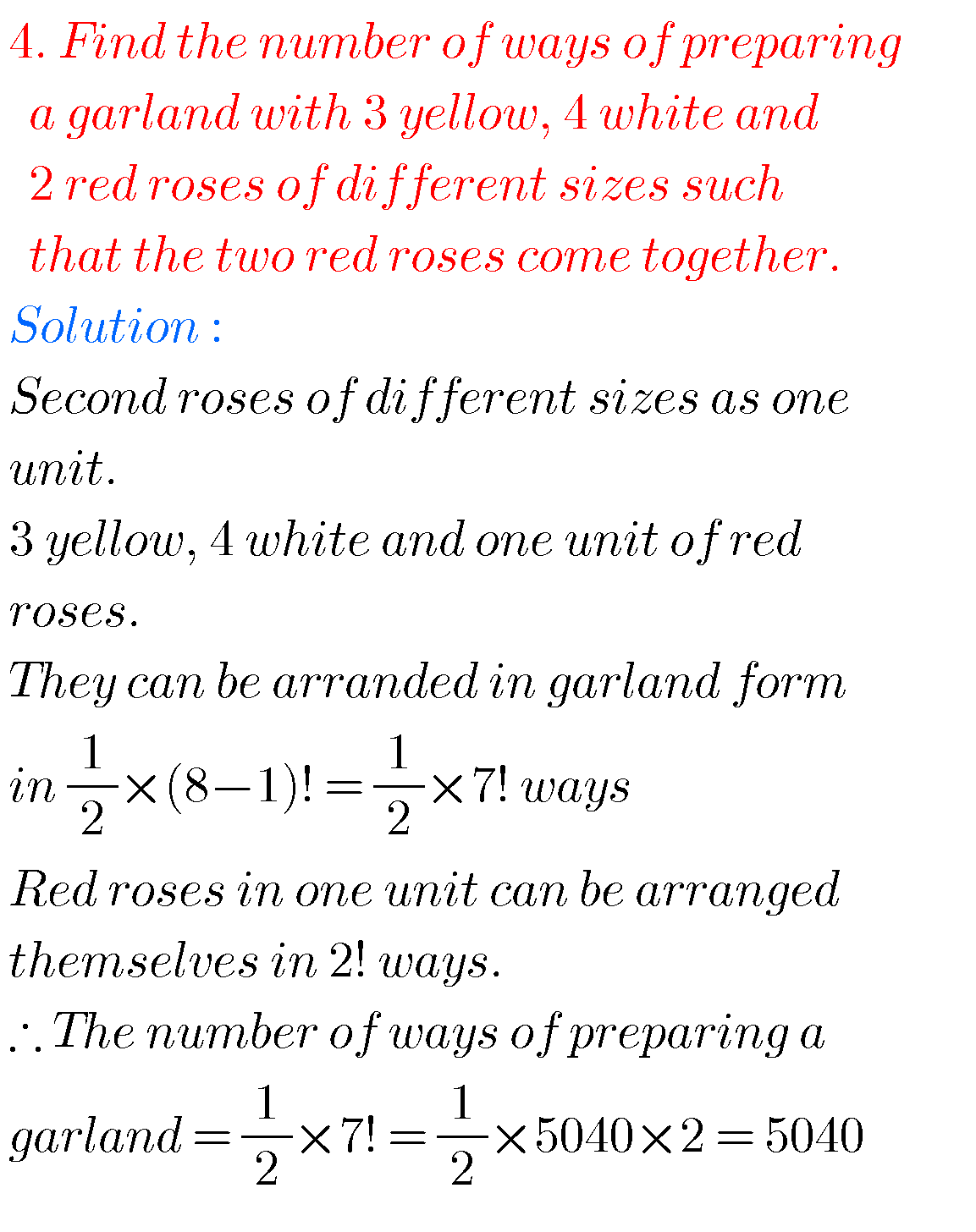###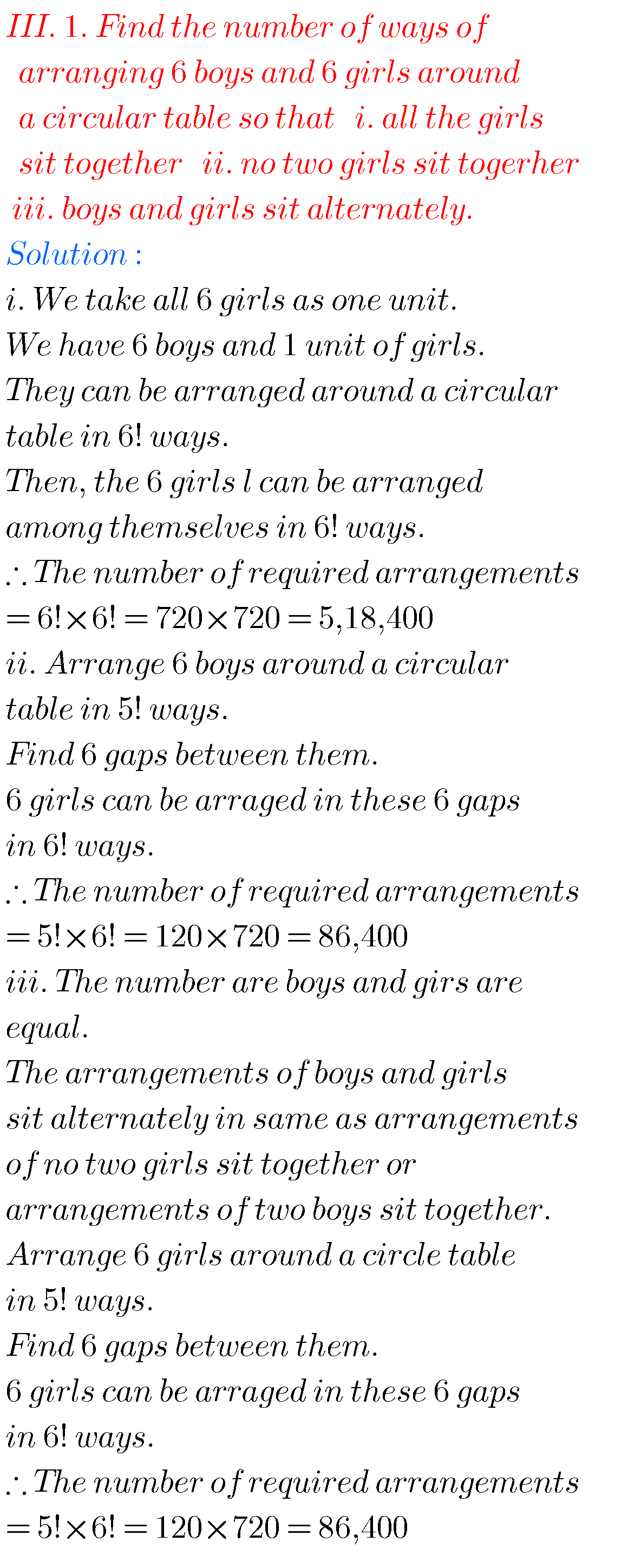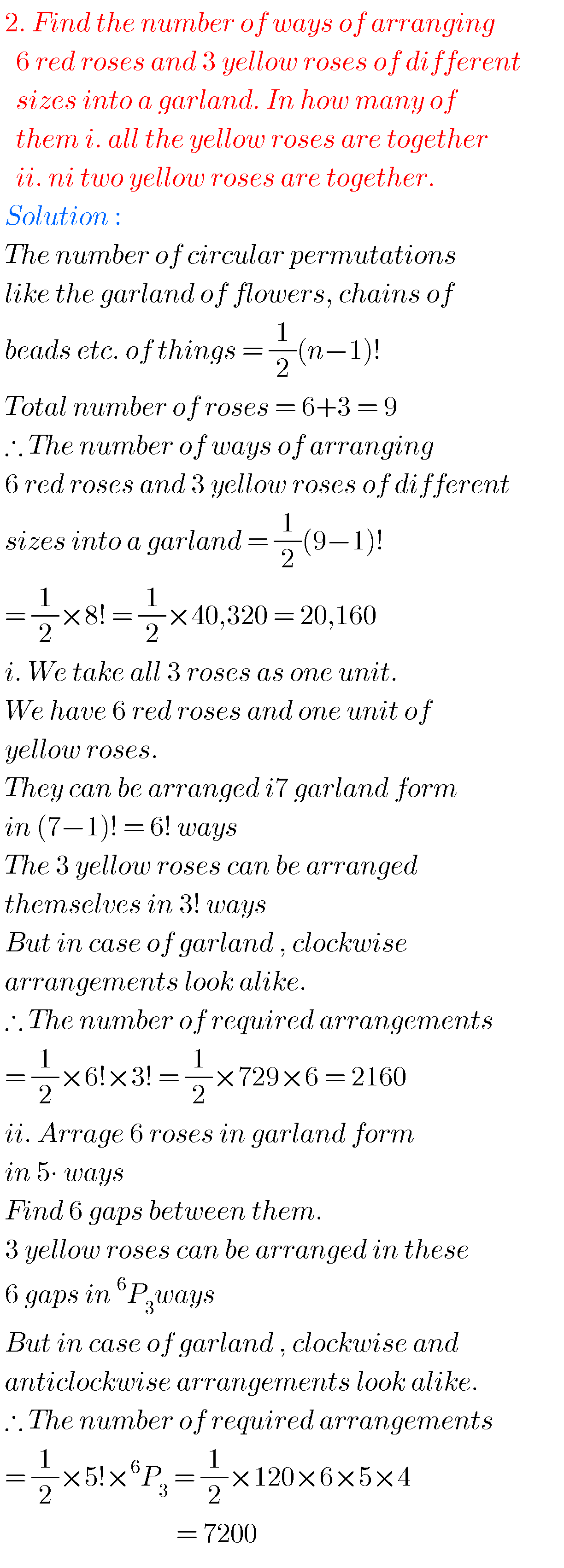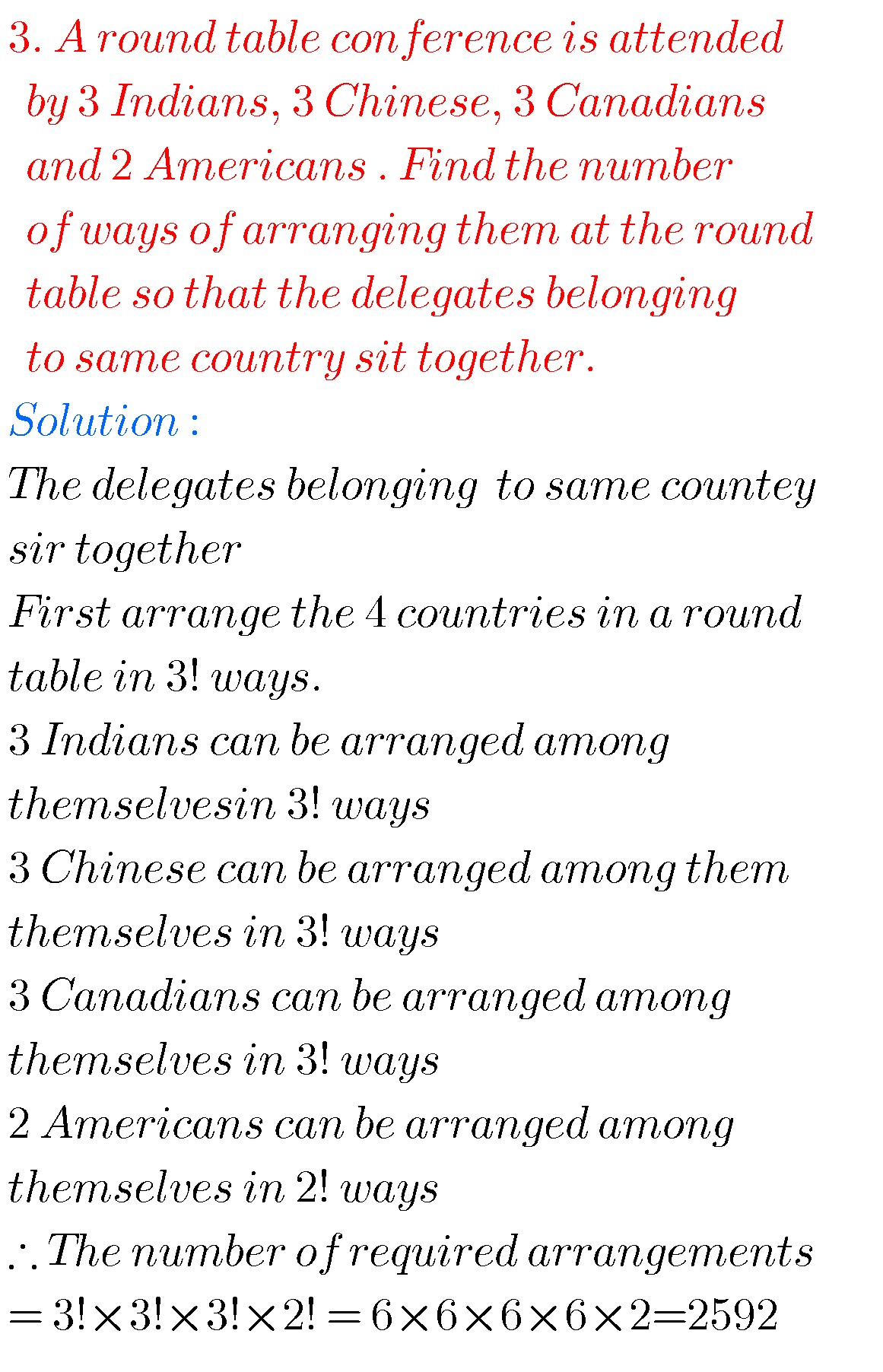### Inter solutions for maths Permutations and Combinations

Exercise 5(d)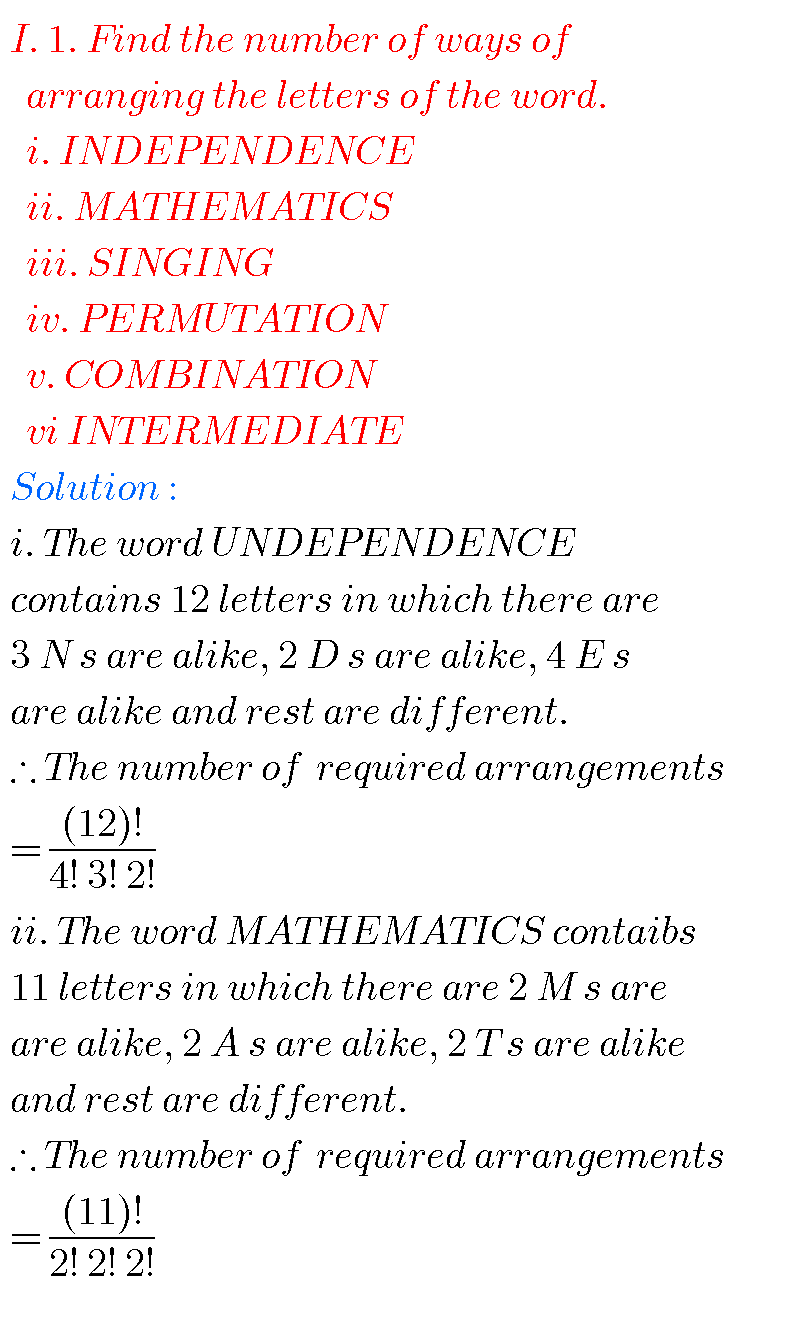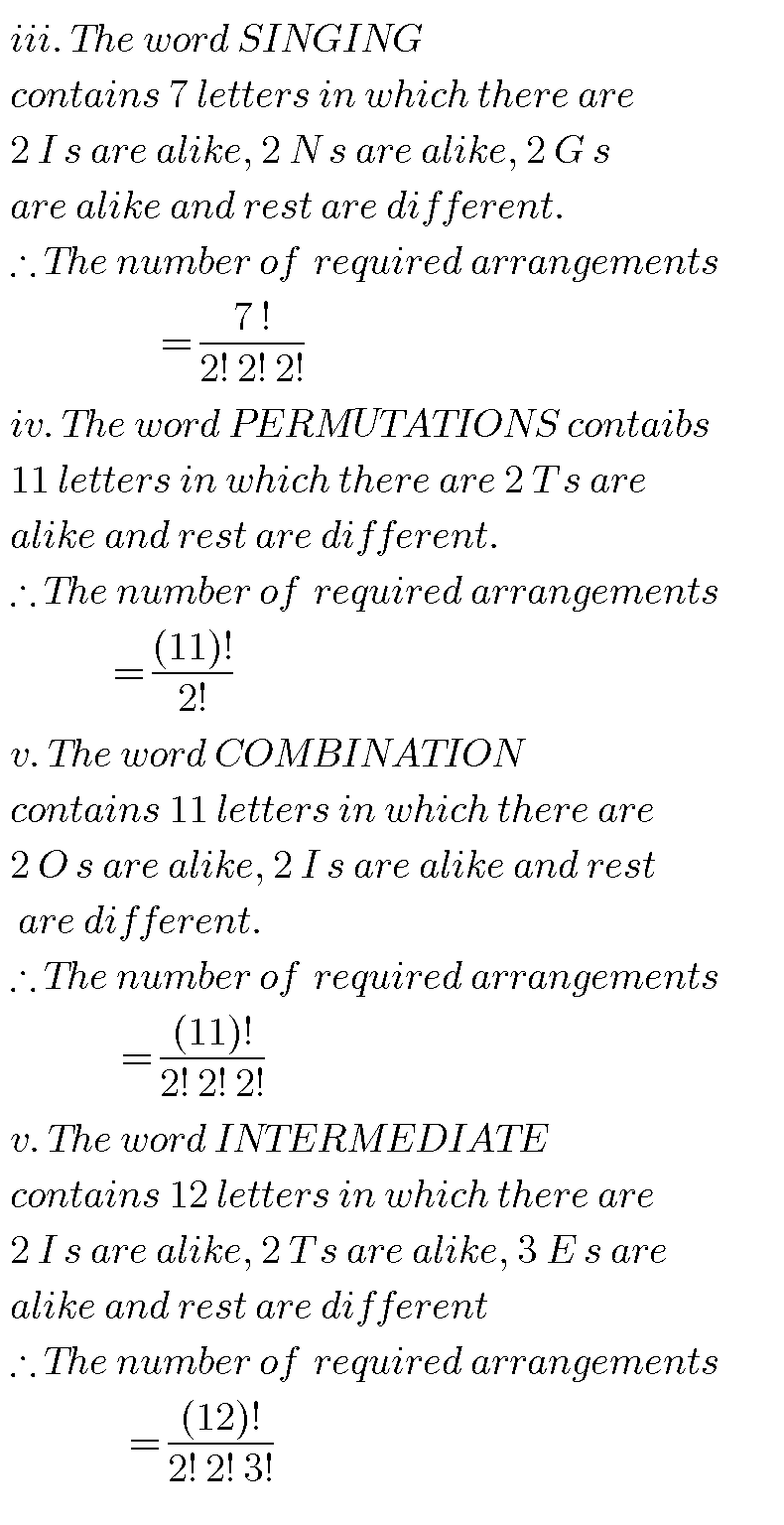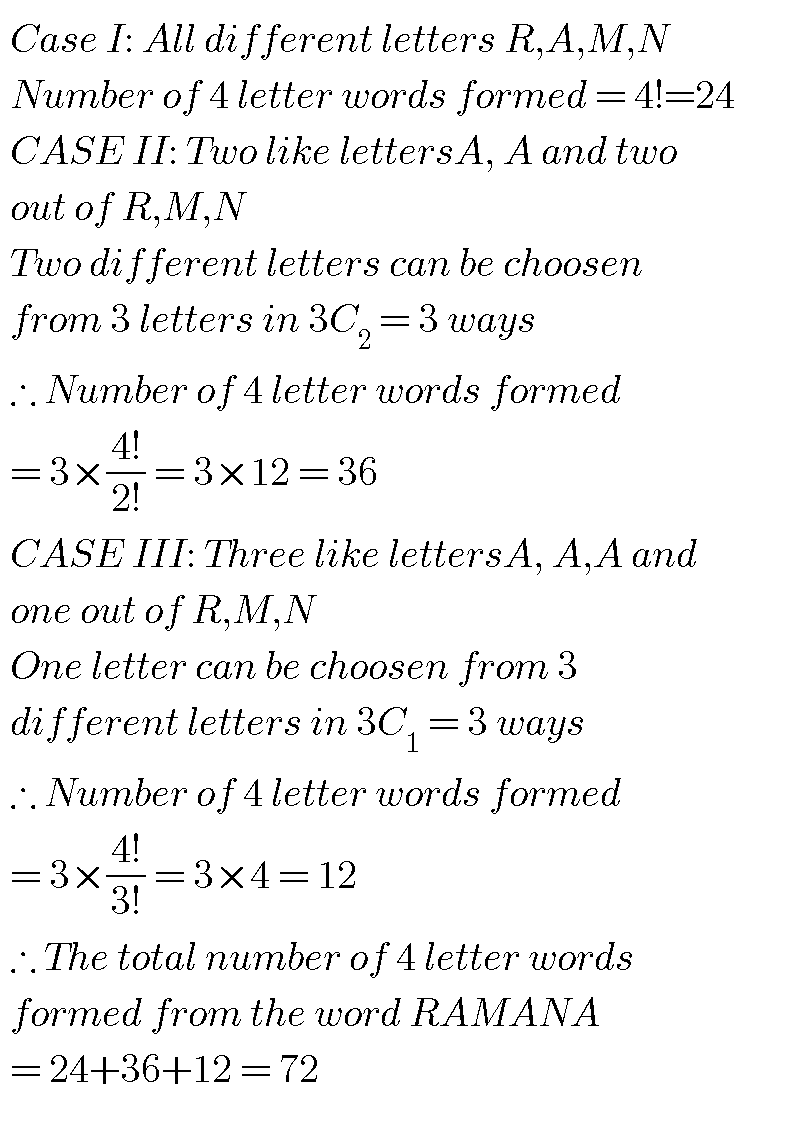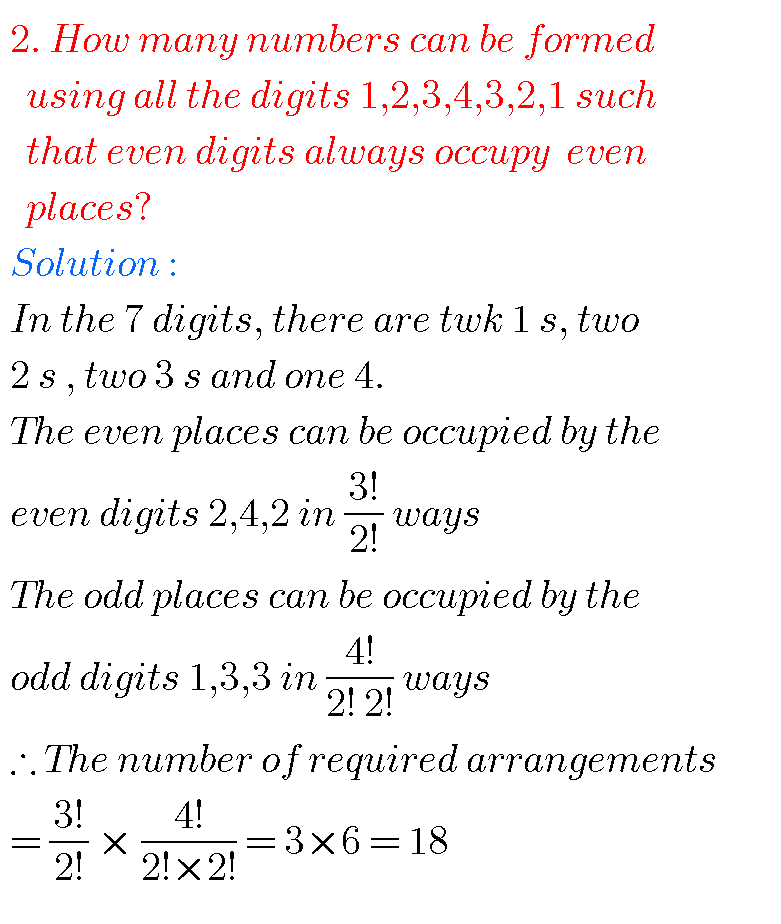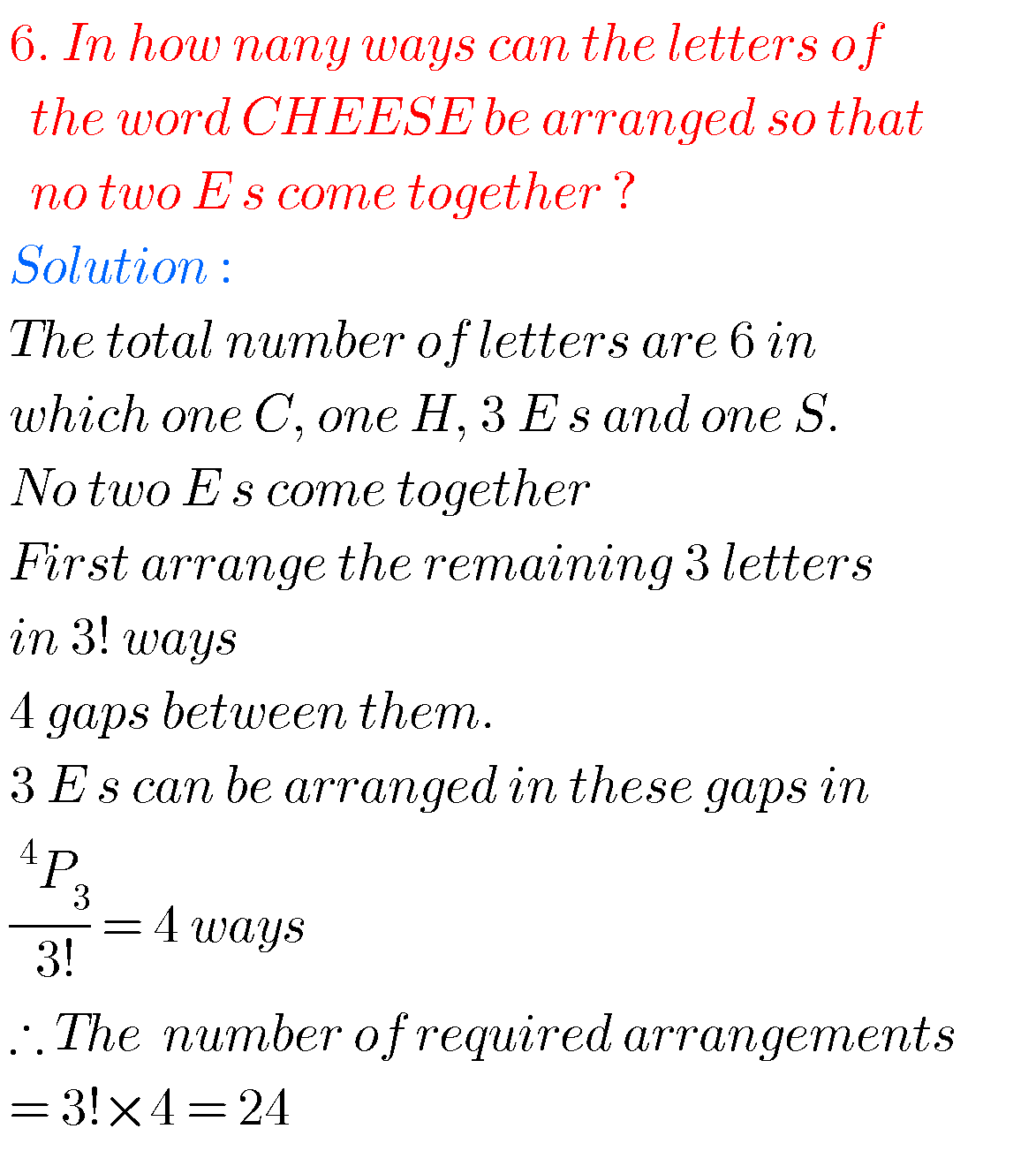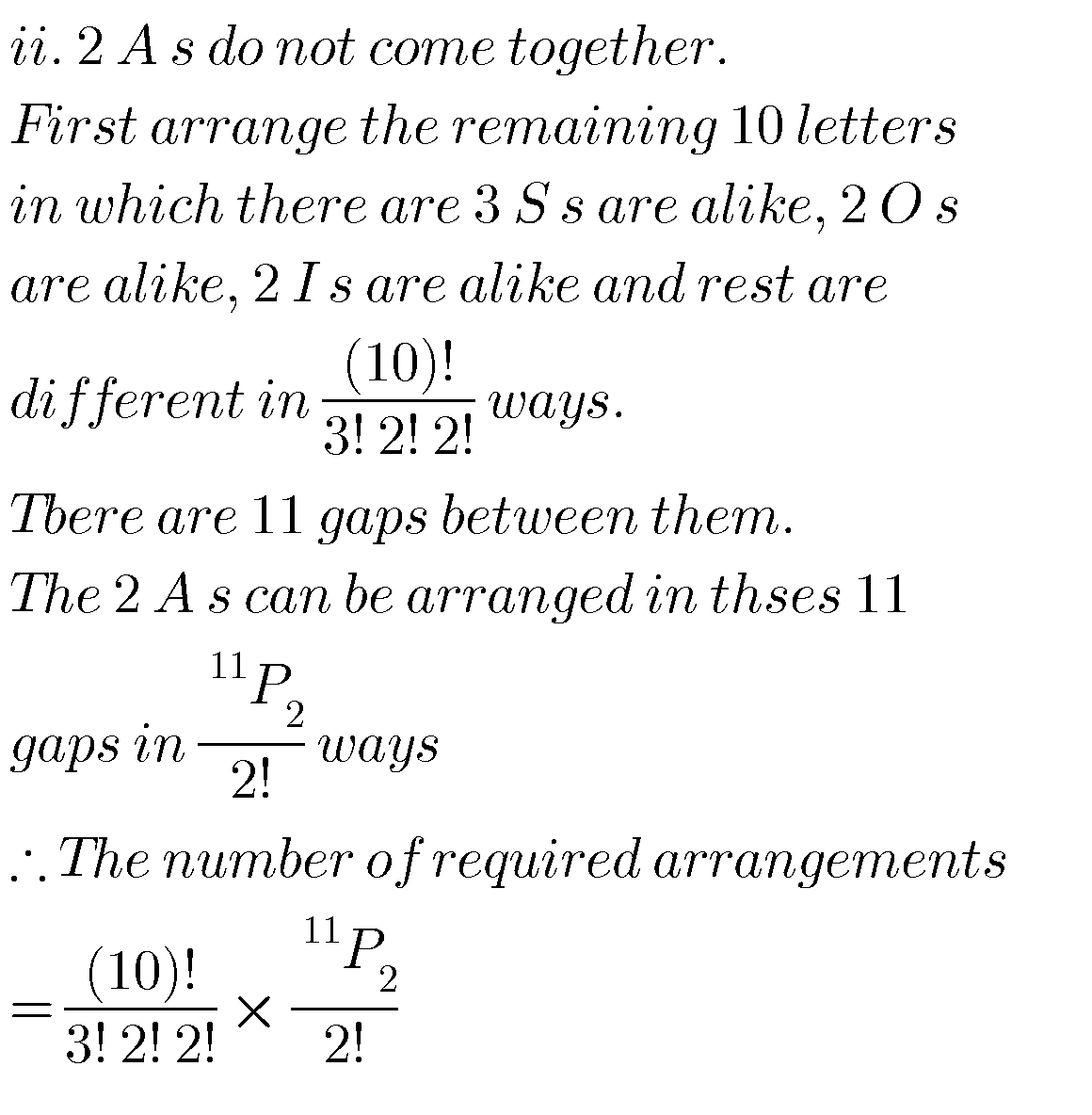M

Inter second year maths Permutations and Combinations solutions

Exercise 5(d)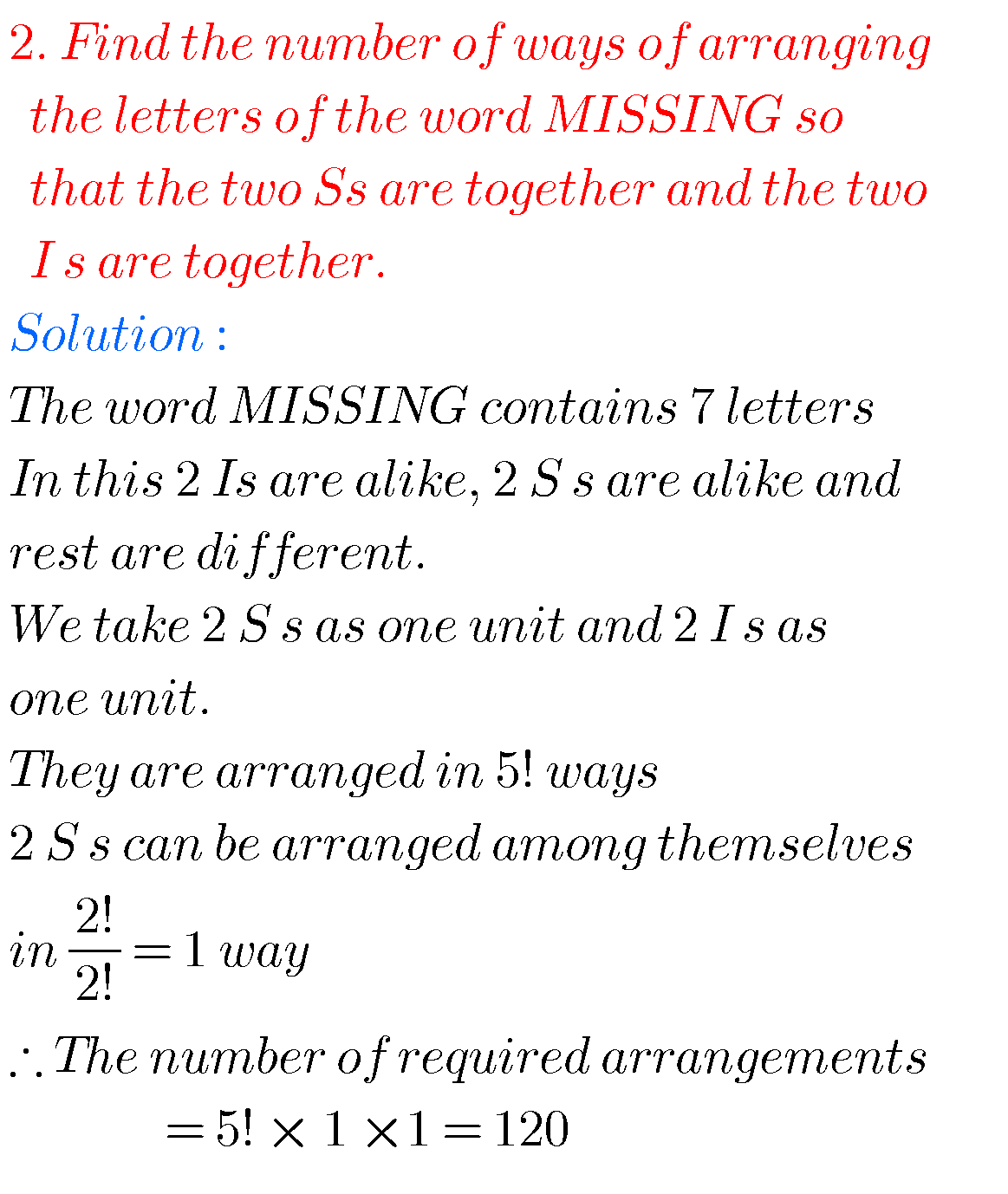## Maths IIA inter solutions for Permutations and Combinations

Exercise 5(e)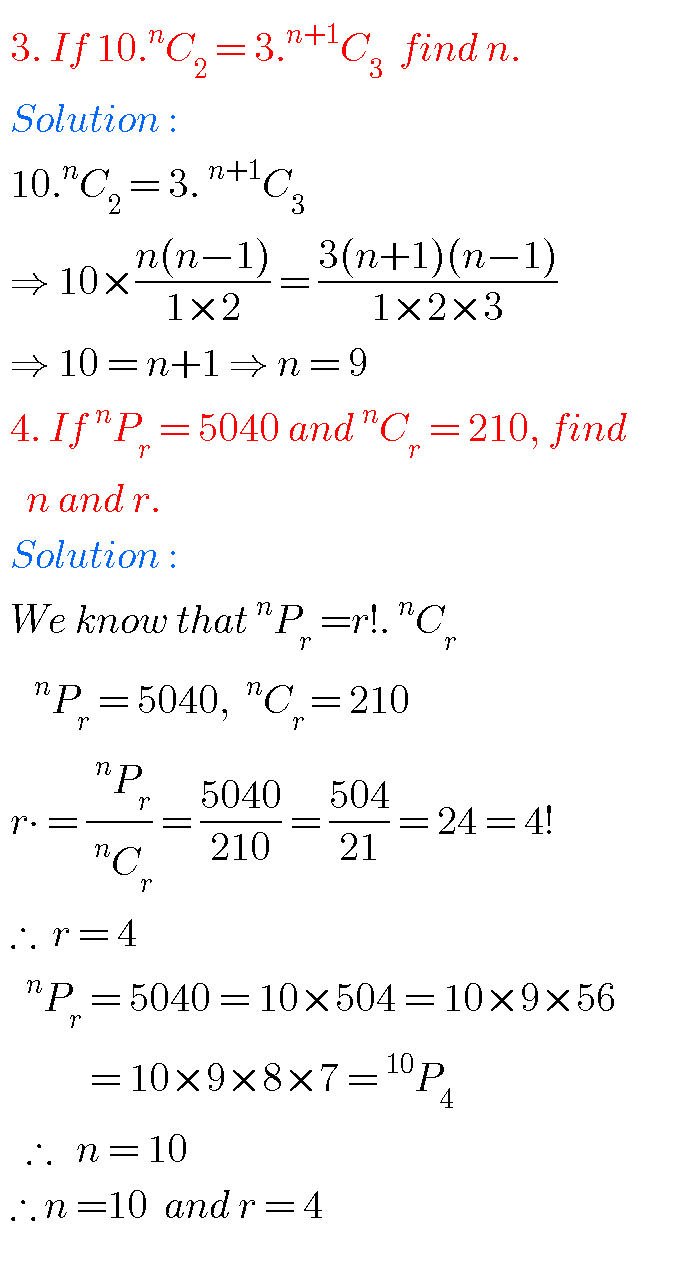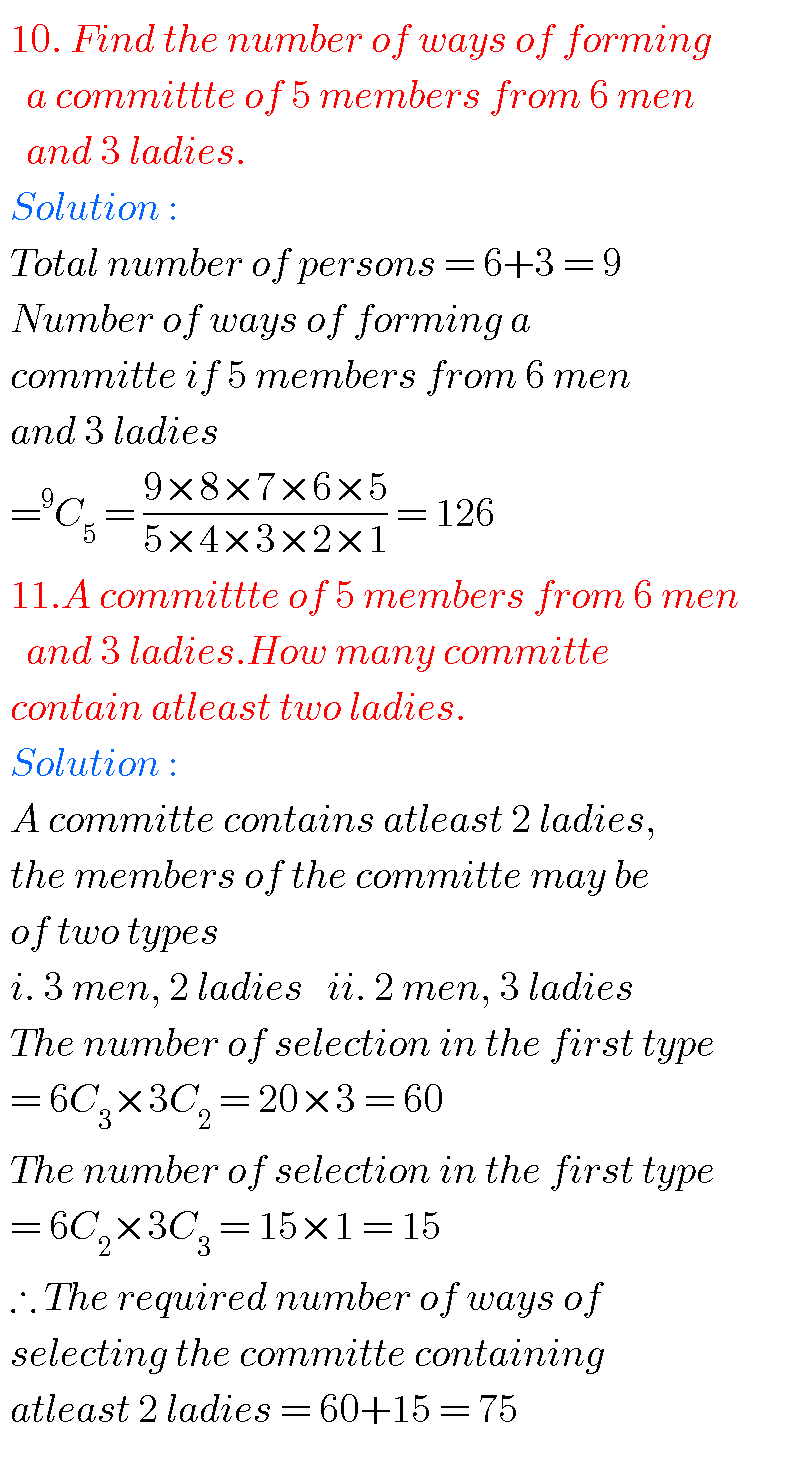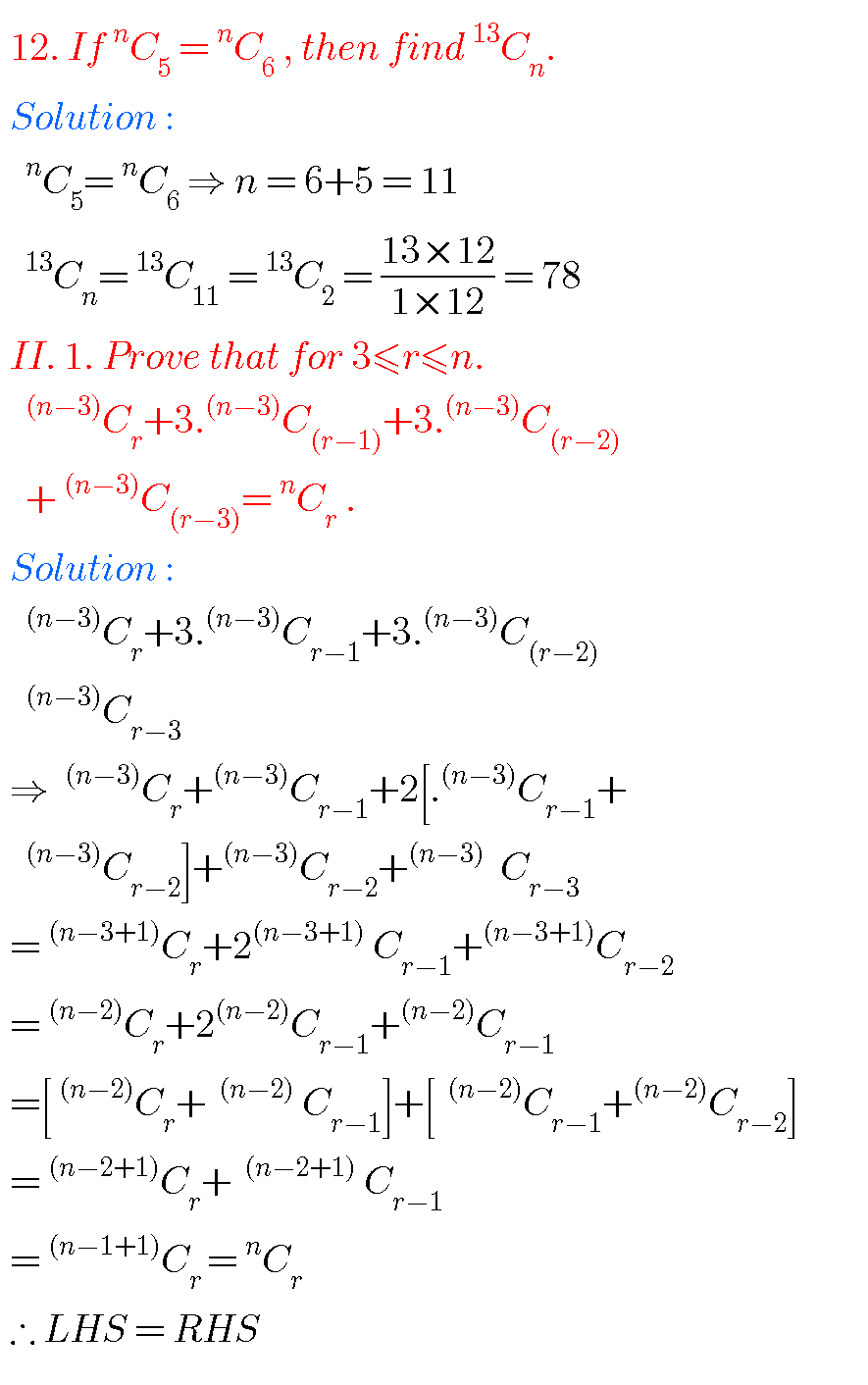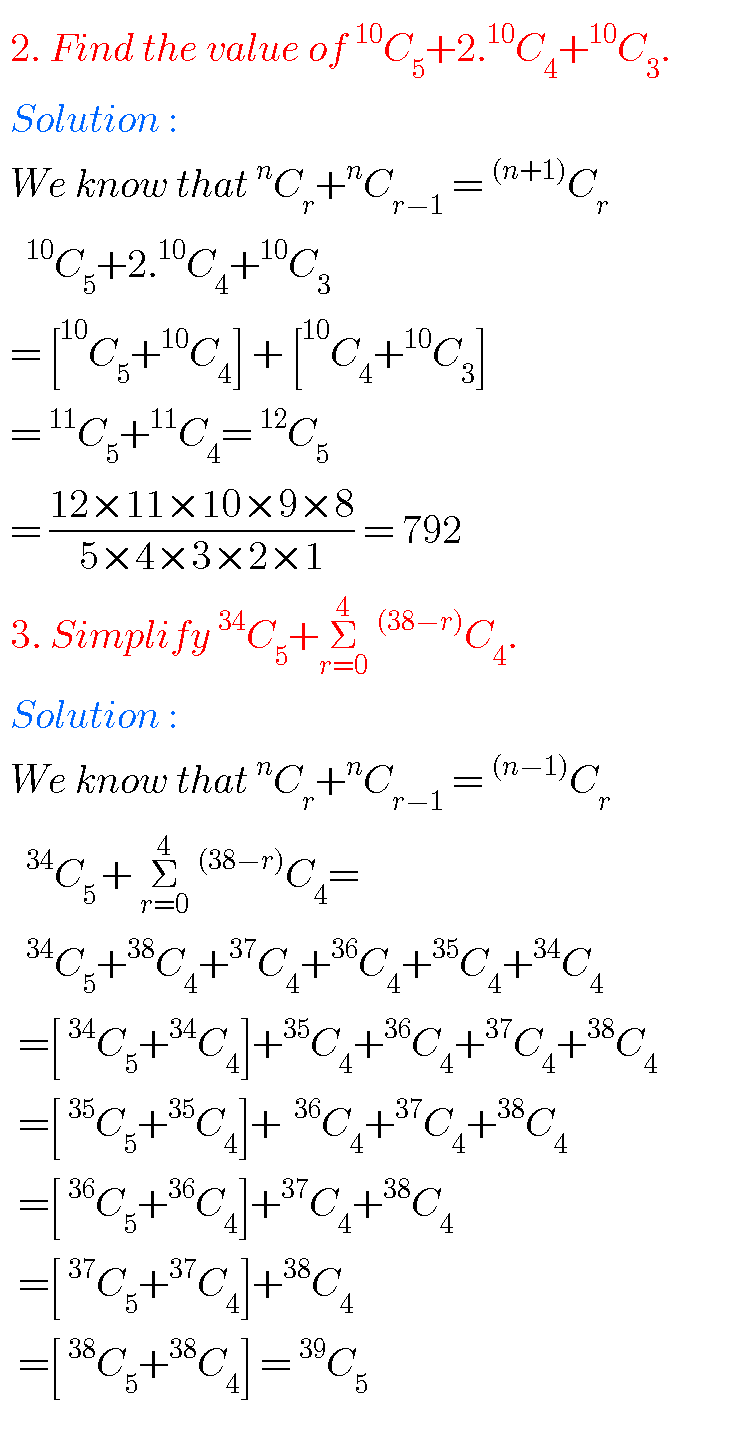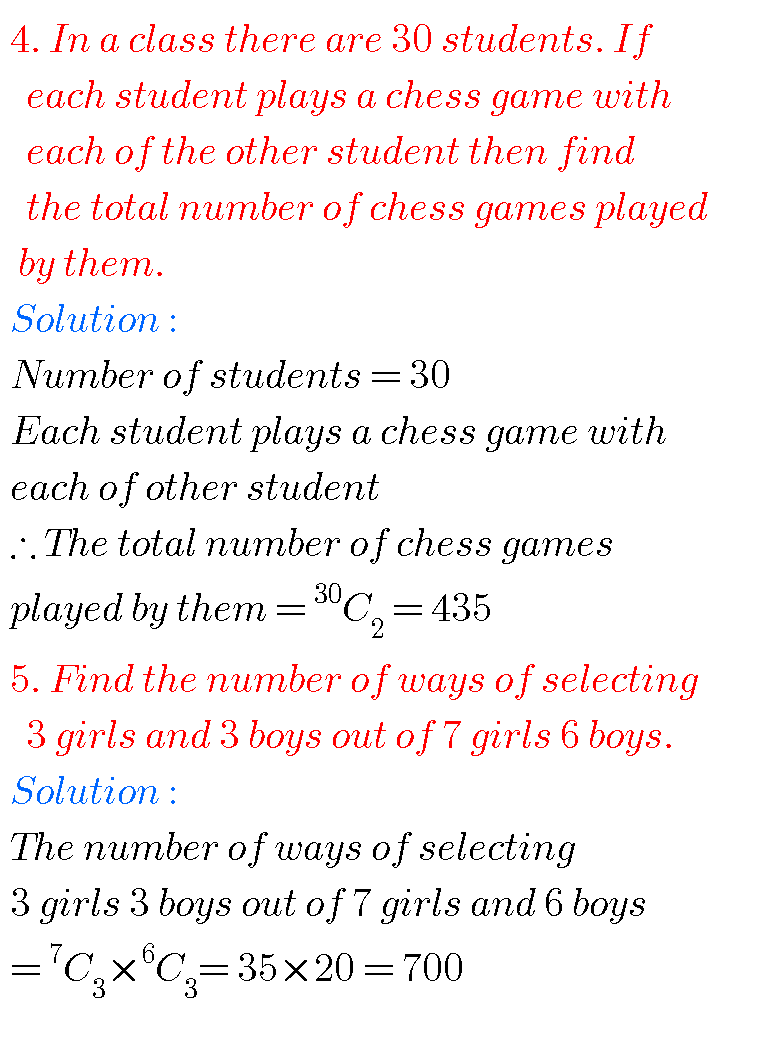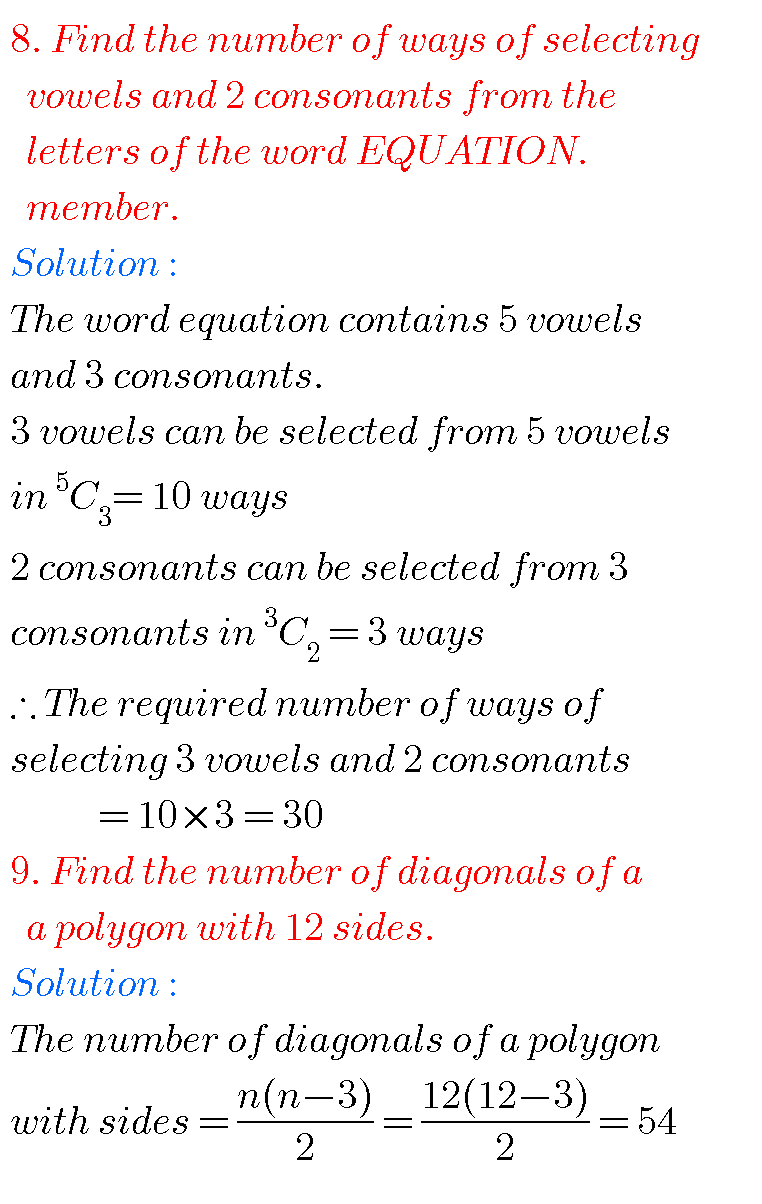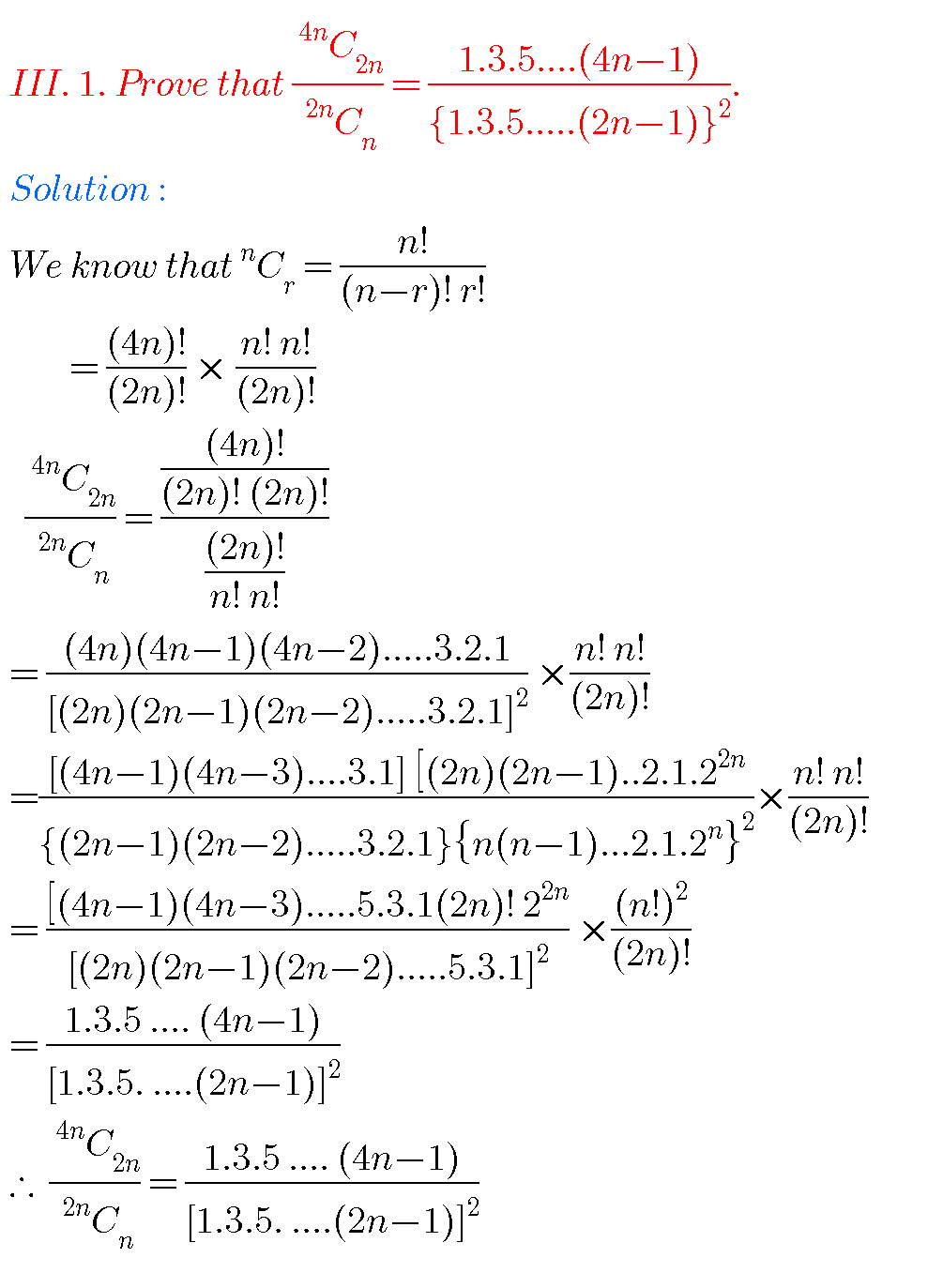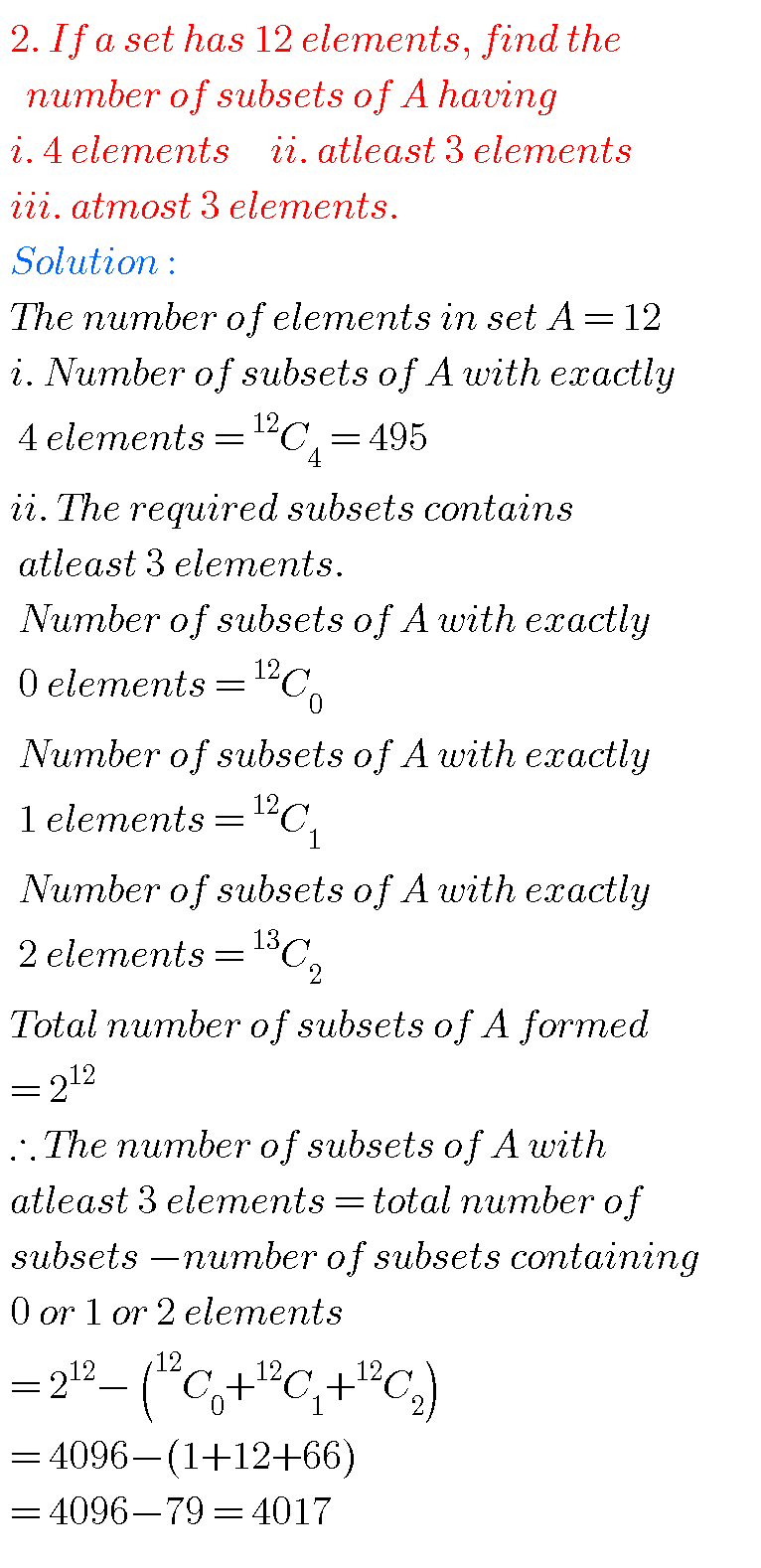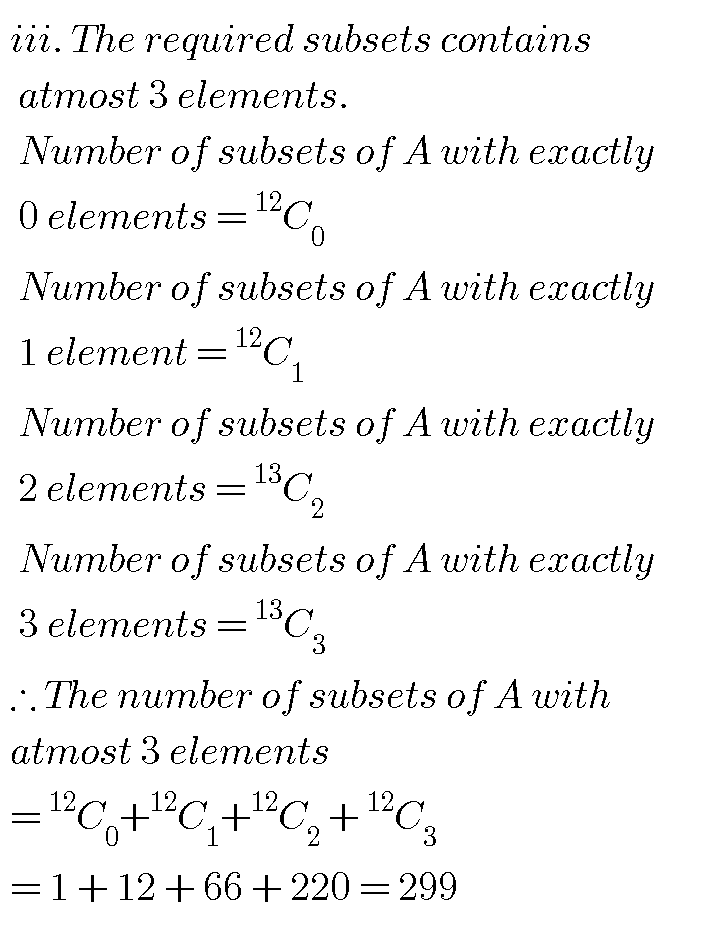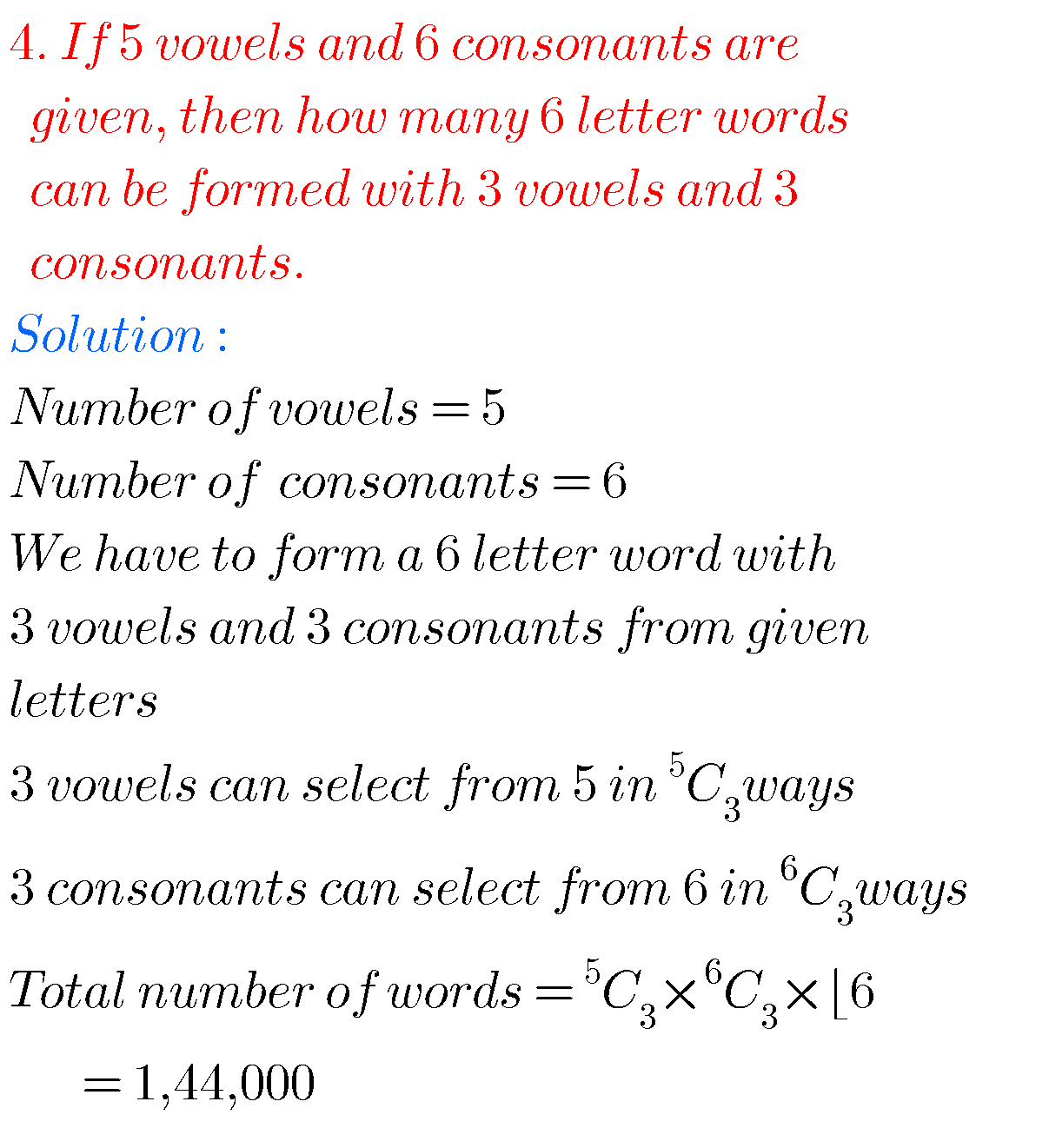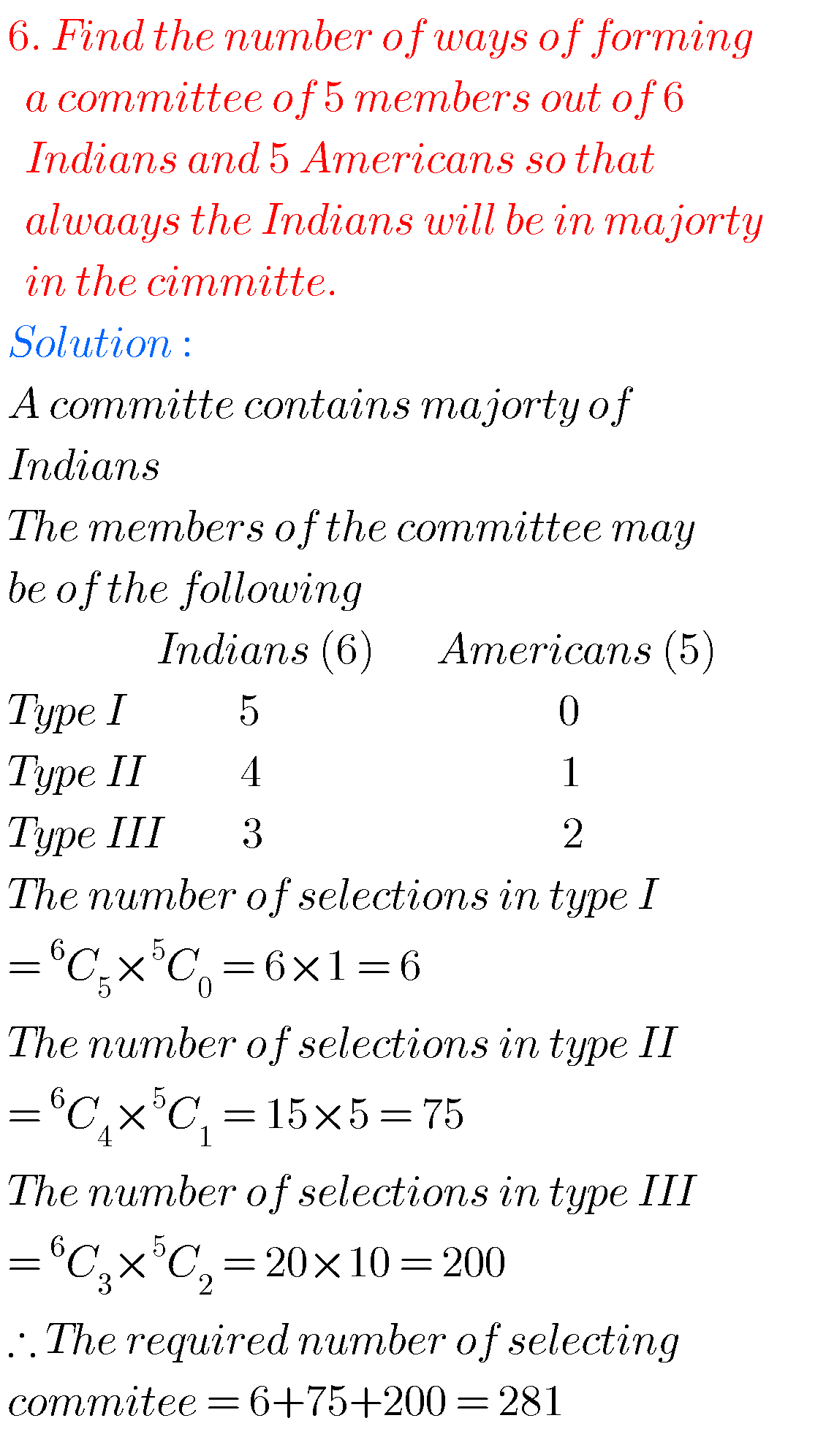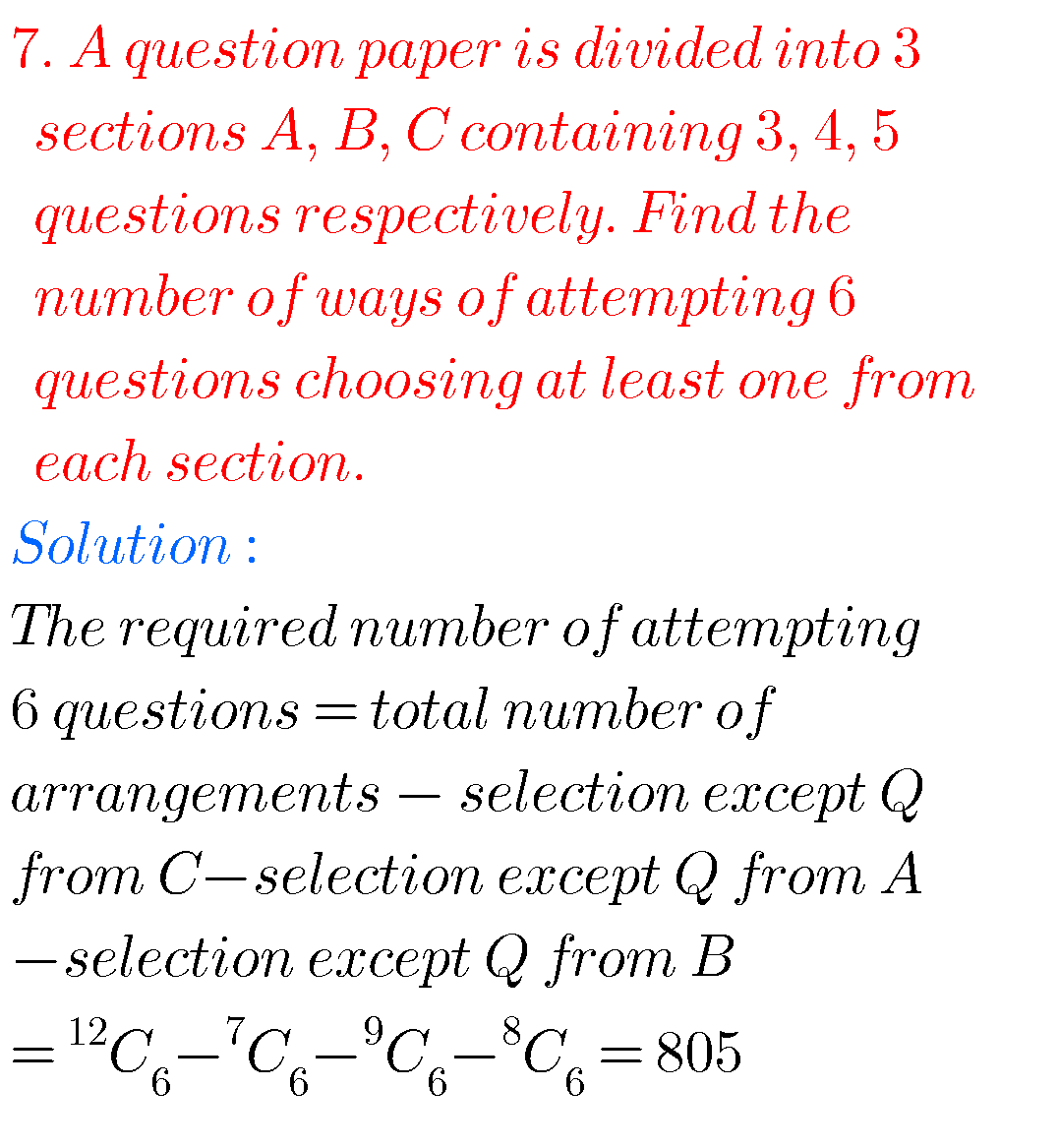Note : Observe the solutions and try them in your own method.

Inter 1a exercise 6(a) solutions

Inter maths 1a exercise 6(b) solutions

class 10 maths polynomials solutions

Ncert maths class 8 exponents and powers

Ncert maths class 7 fractions and decimals

Ncert maths class 6 playing with numbers

ou can see the solutions for text book Maths 1A

Functions

Exercise 1(a)

Exercise 1(b)

Exercise 1(c)

Mathematical Induction

Exercise 2(a)

Matrices

Exercise 3(a)

Exercise 3(b)

Exercise 3(c)

Exercise 3(d)

Exercise 3(e)

Exercise 3(f)

Exercise 3(g)

Exercise 3(h)

Exercise 3(i)

Exercise 4(a)

Exercise 4(b)

Product of Vectors

Exercise 5(a)

Exercise 5(b)

Exercise 5(c)

Trigonometric Ratios up to Transformations

Exercise 6(a)

Exercise 6(b)

Exercise 6(c)

Exercise 6(d)

Exercise 6(e)

Exercise 6(f)

Trigonometric Equations

Exercise 7(a)

Inverse Trigonometric Equations

Exercise 8(a)

Hyperbolic Functions

Exercise 9(a)

Properties of Triangles

Exercise 10(a)

Exercise 10(b)

Maths 1B textbook solutions

Locus

Exercise 1(a)

Transformation of axes

Exercise 2(a)

The Straight Line

Exercise 3(a)
Exercise 3(b)
Exercise 3(c)
Three Dimensional Coordinates

Exercise 5(a)

Exercise 5(b)

Direction Cosines and Direction Ratios

Exercise 6(a)

The Plane

Exercise 7(a)

Limits and Continuity

Exercise 8(a)

Exercise 8(b)

Exercise 8(e)

Applications of Derivaties